# US5764523A - Electronic watt-hour meter - Google Patents

## Info

Publication number
US5764523A
US5764523A US08/295,872 US29587294A US5764523A US 5764523 A US5764523 A US 5764523A US 29587294 A US29587294 A US 29587294A US 5764523 A US5764523 A US 5764523A
Authority
US
United States
Prior art keywords
means
value
ac
quantized
analog
Prior art date
Legal status (The legal status is an assumption and is not a legal conclusion. Google has not performed a legal analysis and makes no representation as to the accuracy of the status listed.)
Application number
US08/295,872
Inventor
Tooru Yoshinaga
Katuhiko Takahashi
Shinzou Tamai
Current Assignee (The listed assignees may be inaccurate. Google has not performed a legal analysis and makes no representation or warranty as to the accuracy of the list.)
Mitsubishi Electric Corp
Original Assignee
Mitsubishi Electric Corp
Priority date (The priority date is an assumption and is not a legal conclusion. Google has not performed a legal analysis and makes no representation as to the accuracy of the date listed.)
Filing date
Publication date
Priority to JP47293 priority Critical
Priority to JP5-000472 priority
Priority to JP5-314689 priority
Priority to JP05314689A priority patent/JP3080207B2/en
Application filed by Mitsubishi Electric Corp filed Critical Mitsubishi Electric Corp
Priority to PCT/JP1994/000008 priority patent/WO1994016334A1/en
Assigned to MITSUBISHI DENKI KABUSHIKI KAISHA reassignment MITSUBISHI DENKI KABUSHIKI KAISHA ASSIGNMENT OF ASSIGNORS INTEREST (SEE DOCUMENT FOR DETAILS). Assignors: TAMAI, SHINZOU, YOSHINAGA, TOORU, TAKAHASHI, KATUHIKO
Application granted granted Critical
Publication of US5764523A publication Critical patent/US5764523A/en
Anticipated expiration legal-status Critical
Application status is Expired - Lifetime legal-status Critical

## Images

••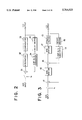•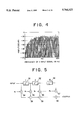•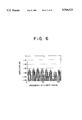•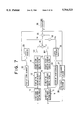•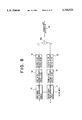•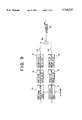•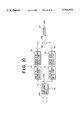•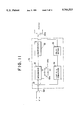•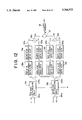•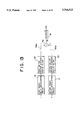•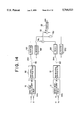•••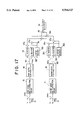•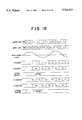•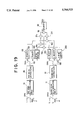•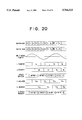••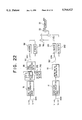•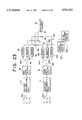•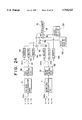•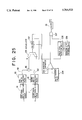••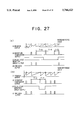•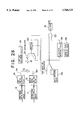•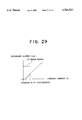•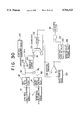•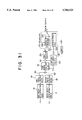•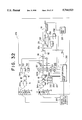•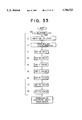•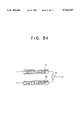## Classifications

• GPHYSICS
• G01MEASURING; TESTING
• G01RMEASURING ELECTRIC VARIABLES; MEASURING MAGNETIC VARIABLES
• G01R21/00Arrangements for measuring electric power or power factor
• G01R21/133Arrangements for measuring electric power or power factor by using digital technique
• G01R21/1331Measuring real or reactive component, measuring apparent energy
• GPHYSICS
• G01MEASURING; TESTING
• G01RMEASURING ELECTRIC VARIABLES; MEASURING MAGNETIC VARIABLES
• G01R21/00Arrangements for measuring electric power or power factor
• G01R21/133Arrangements for measuring electric power or power factor by using digital technique

## Abstract

In a method of calculating an electric power by digitalizing a voltage signal and a current signal of an electronic watt-hour meter and multiplying digitalized signals, precision of an electric power calculation is improved by a circuit arrangement suitable for use in an IC mainly composed of a digital circuit with a small analog scale. A voltage signal and a current signal are inputted into analog to digital conversion means composed of a sigma-delta modulation circuit and the like, respectively, and a moving average of outputs from the analog to digital conversion means is determined by moving average processing means as a low-pass filter and then voltages are multiplied by currents. An analog scale for sigma-delta modification is small. Additionally, the circuit arrangement is reduced by the employment of the low-pass filter due to the use of the moving average. Further, an electric power can be calculated with a high precision.

## Description

TECHNICAL FIELD

The present invention relates to an electronic watt-hour meter for converting analog input signals of a voltage and a current into digital values and processing the digital values.

BACKGROUND ART

FIG. 34 shows a block diagram of a circuit for calculating an electric power of a conventional electronic watt-hour meter.

In FIG. 34, numeral 71 denotes a first successively comparing type A/D converter into which an analog current signal is input, numeral 72 denotes a second successively comparing type A/D converter into which an analog voltage signal is input and numeral 73 denotes a multiplier into which digital data corresponding to a voltage value and a current value from the respective successively comparing type A/D converters 71, 72 is input.

As shown in FIG. 34, the conventional electronic watt-hour meter includes the first and second successively comparing type A/D converters 71, 72 as means for converting analog quantities of a voltage and a current into digital values, and an electronic energy w is obtained by calculating these digital outputs by the multiplier 73.

In general, since the successively comparing type A/D converters 71, 72 quantize an analog input signal to a digital value in such a manner that an output is discretely increased with respect to the analog input signal by the same resolution, a successively comparing type A/D converter with high resolution is needed to obtain absolute precision for a low level input.

For example, when a current signal is supposed to be 1/120 of a maximum input, the following condition must be satisfied in order to keep the accuracy of a quantized current value to 0.5% or less. ##EQU1##

A successively comparing type A/D converter has a S/N ratio represented by

`S/N=6m+1.8 (dB)                                            (2)`

where m is the number of output bits of the successively comparing type A/D converter when the sampling frequency is f2 =2×(signal frequency). Thus, a successively comparing type A/D converter having a resolution of m=15 bits is needed to keep the aforesaid precision of 0.5% or less.

On the other hand, as a method of improving the precision, there is a method of increasing a sampling frequency (fs) of a successively comparing type A/D converter, i.e., a so-called over-sampling method.

For example, when a sampling frequency (fs) is increased to a frequency which is 128 times a sampling frequency (fs =twice a signal frequency) determined by the Nyquist theorem, a quantized noise is dispersed to a wide bandwidth and the level of spectrum of each frequency component is lowered. When it is assumed here that a signal frequency=60 Hz and a sampling frequency (fs)=15.36 KHz, an over-sampling of 128 times is achieved and a noise level of the signal frequency component is improved by about 21 dB, which is equivalent to an improvement of a resolution of a successively comparing type A/D converter by 3-4 bits. In this case, a successively comparing type A/D converter having a resolution of 11-12 bits is needed to keep the precision of 0.5% or less of the quantized current value.

Therefore, a successively comparing type A/D converter having a high resolution and a multiplier to which many bits can be input are needed in conventional devices to obtain a high-precision electronic watt-hour meter, by which the circuit arrangement is of increased complexity and increased cost. In particular, this is very disadvantageous when electronic watt-hour meters composed of monolithic ICs are intended to be produced on a large scale.

SUMMARY OF THE INVENTION

An object of the present invention made to solve the above problems is to provide a high-precision electronic watt-hour meter having a simple circuit arrangement.

The electronic watt-hour meter according to a first aspect of the invention comprises first and second analog to digital conversion means for quantizing an AC current and an AC voltage, respectively, first and second moving average processing means for determining a moving average of each of the quantized AC current and the quantized AC voltage, first multiplication means for multiplying the AC current and the AC voltage each subjected to the moving average processing, and cumulation means for cumulating outputs from the multiplication means.

The electronic watt-hour meter according to a fifth aspect of the invention comprises first analog to digital conversion means for quantizing an AC current, second analog to digital conversion means for quantizing an AC voltage, first and second digital low-pass filters for causing the quantized AC current and AC voltage to pass through a low-pass region, respectively, thinning-out means for thinning out the quantized current and the quantized voltage each having been subjected to the low-pass filtration from the sampled value train thereof at a ratio of 1/m (m: a number larger than the number of delay means of the first and second digital low-pass filters), first multiplication means for multiplying an AC current and an AC voltage from the thinning-out means, and cumulation means for cumulating outputs from the multiplication means.

The electronic watt-hour meter according to an eighth aspect of the invention comprises switching means for successively fetching AC currents and AC voltages of respective multiple-element inputs from multiple-input devices from input AC currents and AC voltages of the corresponding elements at a predetermined cycle, first analog to digital conversion means and second analog to digital conversion means for successively quantizing AC currents and AC voltages of the respective multiple-elements from the switching means, first and second digital low-pass filters for causing the quantized AC currents and AC voltages of the respective multiple-elements to successively pass through a low-pass region, thinning-out means for thinning out the quantized currents and the quantized voltages of the respective multiple-elements each having been subjected to the low-pass filtration from the sampled value train thereof at a ratio of 1/m (m: a number larger than the number of delay means of the first and second digital low-pass filters), calculation means for multiplying AC currents and AC voltages which correspond to the multiple-element input and are supplied from the thinning-out means and determining sums of the multiplication results, and cumulation means for cumulating outputs of the sums.

The electronic watt-hour meter according to a twenty-first aspect of the invention comprises switching means for successively switching at a predetermined cycle and outputting respective currents and voltages entered from a first phase, a second phase and a third phase;

first and second analog to digital conversion means for quantizing AC currents and AC voltages of the respective phases output from the switching means, respectively;

first and second digital low-pass filters for causing the quantized AC currents and the quantized AC voltages of the respective phases to pass through a low-pass region, respectively;

thinning-out means for thinning out the quantized AC currents and the quantized AC voltages of the respective phases each having been subjected to the low-pass filtration from the sampled value train thereof at a ratio of 1/m;

calculation means for multiplying the AC currents of the respective phases from the thinning means by the AC voltages of the respective phases from the thinning means, respectively and determining sums of the multiplication results;

third low-pass filter for causing the outputs of the sums to pass through a low-pass region;

cumulating means for cumulating an output from the third digital low-pass filter; as well as

at least one of the following adjustment means:

(1) balance adjustment means including first and second balance adjustment registers for multiplying quantized electric power values of a second phase and a third phase determined from an AC current and an AC voltage of a usual object to be measured by balance adjustment value B1, B2, respectively and setting a value B2=w01 /w02 to the first balance adjustment register and setting a value B3=W01 /w03 to the second balance adjustment register, where an output from the third digital low-pass filter is represented by w01 at the time when a predetermined analog value of the same phase is input as a current·voltage value of the first phase, the analog value is input as a current·voltage value of the second phase and an output from the third digital low-pass filter at the time is represented by w02, and the analog value is input as a current·voltage value of the first phase and an output from the third digital low-pass filter at the time is represented by w03 ;

(2) rating adjustment means including a register for cumulating outputs from the third digital low-pass filter and an F value setting register for setting a value F=(w01 /reference electric power)×(rated reference value) (where, reference electric power=value produced in calculation determined by reference voltage·reference current, and rated reference value=value in calculation for determining the number of pulses per unit of electric energy (constant)), and sending an output for measuring an electric energy each time the cumulated value exceeds the F value as well as resetting the register;

(4) phase adjustment means including a shift register capable of carrying out the desired number of shifts interposed between the second analog to digital conversion means and the second digital low-pass filter and respective registers P1, P2, P3 for designating the number of shifts of the shift register and carrying out phase adjustment by successively shifting respective phases to values P1, P2, P3 by the shift register in synchronism with the switching means, where an output the third low-pass filter is represented by w0P1 at the time when an analog value having the same effective value as that of the analog value of the (1) and a power factor=1 is input as an input of the first phase and a value P1=K(w01 ×0.5)-w0P1 (K: constant) is set to the P1 shift register,

an output from the third low-pass filter is represented by w0P2 at the time when an analog value having the same effective value as that of the analog value of the (1) and a power factor=1 is input as an input of a second phase and a value P2=K(w01 ×0.5)-w0P2 is set to the P1 shift register, and

an output from the third low-pass filter is represented by w0P3 at the time when an analog value having the same effective value as that of the analog value of the (1) and power factor=1 is input as an input of the second phase and a value P3=K(w01 ×0.5) w0P3 is set to the P1 shift register.

According to the first aspect of the invention, an AC current and an AC voltage as analog values are quantized by the first and second analog to digital conversion means, the quantized signals are subjected to a moving average processing by the first and second moving average processing means, signals corresponding to the current and voltage, respectively are multiplied by the multiplier to obtain signals corresponding to electric power and the signals are cumulated to obtain a high precision electric energy.

According to the fifth aspect of the invention, an AC current is digitized by the first analog to digital conversion means, an AC voltage is digitized by the second analog to digital conversion means, the digitized AC current and AC voltage are caused to pass through a low-pass region by the first and second low-pass filters, respectively. The AC current and AC voltage having been passed through the low-pass region are thinned out from the sampled value train thereof by the thinning-out means at a ratio of 1/m (m: a number larger than the number of delay means of the first and second digital low-pass filters). The AC current and AC voltage from the thinning-out means are multiplied by the first multiplication means, and outputs from the multiplication means are cumulated by the cumulation means to thereby obtain a high-precision electric energy.

According to the eighth aspect of the invention, AC currents and AC voltages of respective multiple-elements are successively fetched at a predetermined cycle from AC currents and AC voltages of the corresponding multiple-elements input by the switching means, the AC currents and AC voltages of the respective elements from the switching means are successively quantized by the first analog to digital conversion means and the second analog to digital conversion means, caused to successively pass through the low-pass region by the first and second digital low-pass filters, and thinned out by the thinning-out means at a ratio of 1/m (m: a number larger than the number of delay means of the first and second digital low-pass filters), AC currents and AC voltages from the thinning-out means which correspond to the multiple-element inputs are multiplied, respectively, sums of the multiplied results are determined by the calculation means and outputs of the sums are cumulated by the cumulation means to obtain an electric energy.

According to the twenty-first aspect of the invention, respective currents and voltages input from a first phase, a second phase and a third phase are successively switched at a predetermined cycle and output by the switching means, and AC currents and AC voltages of the respective phases are quantized by the first and second analog to digital conversion means, respectively. Next, the quantized AC currents and AC voltages are caused to pass through the low-pass region by the first and second digital low-pass filters, the quantized AC currents and the quantized AC voltages of the respective phases each having been subjected to the low-pass filtration are thinned out by the thinning-out means from the sampled value train thereof at a ratio of 1/m, the AC currents and the AC voltages of the respective phases from the thinning-out means are multiplied, respectively and the sums of the results of multiplication are determined by the calculation means, and outputs of the sums are caused to pass through the low-pass region by the third low-pass filter and cumulated by the cumulation means. At the same, the result of cumulation is adjusted by using at least one of the following adjustment means (1), (2), (3), and (4):

(1) balance adjustment means including first and second balance adjustment registers for multiplying quantized electric power values of a second phase and a third phase determined from an AC current and an AC voltage of a usual object to be measured by balance adjustment value B1, B2, respectively and setting a value B2=w01 /w02 to the first balance adjustment register and setting a value B3=w01 /w03 to the second balance adjustment register, where an output from the third digital low-pass filter is represented by w01, at the time when a predetermined analog value of the same phase is input as a current·voltage value of the first phase, the analog value is input as a current·voltage value of the second phase and an output from the third digital low-pass filter at the time is represented by w02, and the analog value is input as a current·voltage value of the first phase and an output from the third digital low-pass filter at the time is represented by w03 ;

(2) rating adjustment means including a register for cumulating outputs from the third digital low-pass filter and an F value setting register for setting a value F=(w01 /reference electric power)×rated reference value (where, reference electric power=value produced in calculation determined by reference voltage·reference current, and rated reference value=value in calculation for determining the number of pulses per unit of electric energy (constant)), and sending an output for measuring an electric energy each time the cumulated value exceeds the F value as well as resetting the register;

(4) phase adjustment means including a shift register capable of carrying out the desired number of shifts interposed between the second analog to digital conversion means and the second digital low-pass filter and respective registers P1, P2, P3 for designating the number of shifts of the shift register and carrying out phase adjustment by successively shifting respective phases to values P1, P2, P3 by the shift register in synchronism with the switching means, where an output from the third low-pass filter is represented by w0P1 at the time when an analog value having the same effective value as that of the analog value of the (1) and a power factor=1 is input as an input of the first phase and a value P1=K(w01 ×0.5)-w0P1 (K: constant) is set to the P1 shift register,

an output from the third low-pass filter is represented by w0P2 at the time when an analog value having the same effective value as that of the analog value of the (1) and a power factor=1 is input as an input of a second phase and a value P2=K(w01 ×0.5)-w0P2 is set to the P1 shift register, and

an output from the third low-pass filter is represented by w0P3 at the time when an analog value having the same effective value as that of the analog value of the (1) and power factor=1 is input as an input of the second phase and value P3=K (w01 ×0.5)-w0P3 is set to the P1 shift register.

BRIEF DESCRIPTION OF THE DRAWINGS

FIG. 1 shows a block diagram of an electric power calculation unit of an electronic watt-hour meter according to an embodiment 1 of the present invention;

FIG. 2 shows a block diagram of a primary sigma-delta modulation circuit by way of example;

FIG. 3 shows a block diagram of a secondary sigma-delta modulation circuit by way of example;

FIG. 4 is a graph showing a distribution of quantized noise spectrum of the secondary sigma-delta modulation circuit by way of example;

FIG. 5 shows a block diagram of a digital filter constituting a low-pass filter by way of example;

FIG. 6 is a graph showing a quantized noise spectrum having been subjected to processing by a moving average digital filter;

FIG. 7 shows a block diagram of an electric power calculation unit of an electronic watt-hour meter according to an embodiment 2 of the present invention;

FIG. 8 shows a block diagram of an electric power calculation unit of an electronic watt-hour meter according to an embodiment 3 of the present invention;

FIG. 9 shows a block diagram of an electric power calculation unit of an electronic watt-hour meter according to an embodiment 4 of the present invention;

FIG. 10 shows a block diagram of an electric power calculation unit of an electronic watt-hour meter according to an embodiment 5 of the present invention;

FIG. 11 shows a block diagram of a primary sigma-delta modulation circuit used for embodiments 5 and 6 of the present invention by way of example;

FIG. 12 shows a block diagram of an electric power calculation unit of an electronic watt-hour meter according to an embodiment 6 of the present invention;

FIG. 13 shows a block diagram of an electric power calculation unit of an electronic watt-hour meter according to an embodiment 7 of the present invention;

FIG. 14 shows a block diagram of an electric power calculation unit of an electronic watt-hour meter according to an embodiment 8 of the present invention;

FIG. 15 shows a diagram explaining operation of FIG. 14;

FIG. 16 shows a diagram explaining operation of FIG. 14;

FIG. 17 shows a block diagram of an electric power calculation unit of an electronic watt-hour meter according to an embodiment 9 of the present invention;

FIG. 18 shows a diagram explaining operation of FIG. 17 and FIG. 19;

FIG. 19 shows a block diagram of an electric power calculation unit of an electronic watt-hour meter according to an embodiment 10 of the present invention;

FIG. 20 shows a diagram explaining operation of an embodiment 11 of the present invention;

FIG. 21 shows a block diagram of an electric power calculation unit of an electronic watt-hour meter according to an embodiment 12 of the present invention;

FIG. 22 shows a block diagram of an electric power calculation unit of an electronic watt-hour meter according to an embodiment 13 of the present invention;

FIG. 23 shows a block diagram of an electric power calculation unit of an electronic watt-hour meter according to an embodiment 14 of the present invention;

FIG. 24 shows a block diagram of an electric power calculation unit of an electronic watt-hour meter according to an embodiment 15 of the present invention;

FIG. 25 shows a block diagram of an electric power calculation unit of an electronic watt-hour meter according to an embodiment 16 of the present invention;

FIG. 26 shows a block diagram of an electric power calculation unit of an electronic watt-hour meter according to an embodiment 17 of the present invention;

FIG. 27 shows a diagram explaining operation of FIG. 25 and FIG. 26;

FIG. 28 shows a block diagram of an electric power calculation unit of an electronic watt-hour meter according to an embodiment 18 of the present invention;

FIG. 29 is a graph showing output characteristics of a CT;

FIG. 30 shows a block diagram of an electric power calculation unit of an electronic watt-hour meter according to an embodiment 19 of the present invention;

FIG. 31 shows a block diagram of an electric power calculation unit of an electronic watt-hour meter according to an embodiment 20 of the present invention;

FIG. 32 shows a block diagram of an electric power calculation unit of an electronic watt-hour meter according to an embodiment 21 of the present invention;

FIG. 33 is a flowchart showing adjustment operation of FIG. 32; and

FIG. 34 shows a block diagram of an electric power calculation unit of a conventional electronic watt-hour meter.

DETAILED DESCRIPTION OF THE INVENTION

Embodiment 1

An embodiment of the present invention will be described below with reference to the drawings. In FIG. 1, numeral 10 denotes a first sigma-delta modulation circuit to which an analog current signal i is input and numeral 11 denotes a second sigma-delta modulation circuit to which an analog voltage signal v is input. Numeral 12 denotes a first 16-tap moving average digital filter for determining a moving average at 16 points (hereinafter, referred to as 16 taps) of an output from the first sigma-delta modulation circuit 16. Numeral 14 denotes a second 16-tap moving average digital filter connected to the first moving average digital filter 12, numeral 13 denotes a third 16-tap moving average digital filter for determining a moving average at 16 taps of an output from the second sigma-delta modulation circuit 11, numeral 15 denotes a fourth 16-tap moving average digital filter connected to the third 16-tap moving average digital filter 13. First moving average processing means is composed of the first and second 16-tap moving average digital filters 12, 14 and second moving average processing means is composed of the third and fourth 16-tap moving average digital filters 13, 15.

Numeral 16 denotes a first one cycle moving average digital filter connected in parallel with the first 16-tap moving average digital filter 12. Numeral 17 denotes a second one cycle moving average digital filter connected in parallel with the third 16-tap moving average digital filter 13. First and second integration means are composed of the first and second one cycle moving average digital filters 16, 17. Numeral 18 denotes a first multiplier to which only one piece of data is input which is thinned out through 1/8 thinning-out means 141, 151 from respective eight pieces of data output from the second and fourth 16-tap moving average digital filters 14, 15. Numeral 19 denotes a second multiplier to which only one piece of data is input which is thinned out through 1/8 thinning-out means 161, 171 from respective eight pieces of data output from the first and second one cycle moving average digital filters 16, 17. Numeral 20 denotes a subtracter which calculates a difference between an output from the first multiplier 18 and an output from the second multiplier 19 to calculate electric power data w. Numeral 30 denotes a counter for integrating the electric power data.

FIG. 2 shows an internal arrangement of a primary sigma-delta modulation circuit constituting the first and second sigma-delta modulation circuits 10, 11 in FIG. 1.

In FIG. 2, an input X(z) is input into an adder 31 in a unit of a sampling frequency (fs). An output from the adder 31 is supplied to an integrator 32 and an output from the integrator 32 is output from a comparator 33 as 1-bit logic data Y(z). The output data is fed back to the adder 31 by a one bit D/A converter 34 through delay means 35. The above arrangement is referred to as the primary sigma-delta modulation circuit.

The primary sigma-delta modulation circuit shown in FIG. 2 has an input/output relation expressed by the following formula (3).

`Y(z)=X(z)+(1-Z.sup.-1) Q(z)                                (3)`

where Q(z) is a noise produced by quantization.

As shown in formula (3), a sigma-delta modulated input signal X(z) appears at an output Y(z) as it is and is further added with a noise Q(z) to form an output data.

Although the aforesaid is an example of the primary sigma-delta modulation circuit, a secondary sigma-delta modulation circuit shown in FIG. 3 may constitute the first and second sigma-delta modulation circuits 10, 11 shown in FIG. 1. In the case of the secondary sigma-delta modulation circuit shown in FIG. 3, adders 41, 46 and integrators 42, 47 are arranged as two stages, respectively and other components are the same as those shown in the primary sigma-delta modulation circuit shown in FIG. 2.

The secondary sigma-delta modulation circuit shown in FIG. 3 has an input/output relation expressed by the following formula (4).

`Y(z)=X(z)+(1-Z.sup.-1).sup.2 Q(z)                          (4)`

where Q(z) is a noise produced by quantization.

An input signal X(z) appears at an output Y(z) as it is similar to the primary sigma-delta modulation circuit also in the formula (4) and is further added with a noise Q(z) to form an output data. The formula (3) is different from the formula (4) only in a distribution of a quantized noise caused by the noise (Q(z)) shown in the second term of the respective formulas.

FIG. 4 shows a distribution of spectrum of a quantized noise caused by a modulating operation of the secondary sigma-delta modulation circuit. As shown in FIG. 4, a low frequency region has a small amount of a quantized noise and a high frequency region has a large amount of quantized noise. Although the aforesaid primary sigma-delta modulation circuit shown in FIG. 2 also shows a similar distribution of a quantized noise, the secondary sigma-delta modulation circuit of FIG. 3 has a feature that the low frequency region has a smaller amount of a quantized noise.

Thus, in the embodiment I of the present invention in FIG. 1, operation will be described supposing that the sampling frequency is fs=122.88 KHz and the secondary sigma-delta modulation circuit shown in FIG. 3 is used as the first and second sigma-delta modulation circuits 10, 11.

As described above, outputs from the first and second sigma-delta modulation circuits 10, 11 are formed by adding a quantized noise to input signals. The outputs are in the form of 1-bit serial logic data with a sampling rate of 122.88 KHz. Thus, the first and third 16-tap moving average digital filters 12, 13 shown in FIG. 1 serve as low-pass filters for attenuating a quantized noise in the high frequency region. As shown in FIG. 5, a digital filter is usually composed of delay means 51, 52, 53, multipliers 54, 55, 56, 57 and adders 58, 59, 60. Although the digital filter shown in FIG. 5 includes coefficients ao, a1, a2, . . . a15 as elements for determining a type of a low-pass filter, a moving average digital filter includes a coefficients a0 =a1 =a2 =. . . a15 =1. Therefore, the moving average digital filter can be realized by a very simple arrangement because the multipliers 54, 55, 56, 57 shown in FIG. 5 are not needed.

The first and second 16-tap moving average digital filters 12, 14 having the arrangement shown in FIG. I can be also realized as a whole by the digital filter shown in FIG. 5 (coefficient an is not only 1). In this case, the first and second 16-tap moving average digital filters 12, 14 are expressed as follows.

`H(z)=(1+Z.sup.-1 + . . . +Z.sup.-15)(1+Z.sup.-1 + . . . +Z.sup.-15)`

The following formula can be obtained by developing the above formula.

`H(z)=(1+2Z.sup.-1 +3Z.sup.-2, . . . +3Z.sup.-28 +2Z.sup.-29 +Z.sup.-30)`

More specifically, the digital filter shown in FIG. 5 which has the coefficient an of (1, 2, 3, . . . 3, 2, 1) and 31 taps is equivalent with a two-staged moving average digital filter. This is also applied to the third and fourth 16-tap moving average digital filters 13, 15 shown in FIG. 1 in the same way.

FIG. 6 shows a distribution of a spectrum of a quantized noise of an output having passed through the first and third 16-tap moving average digital filters 12, 13 and further the second and fourth 16-tap moving average digital filters 14, 15. Output data having a 8-bit width of the second 16-tap moving average digital filter 14 corresponding to an analog current signal i and output data having a 8-bit width of the fourth 16-tap moving average digital filter 15 corresponding to an analog voltage signal v are used by the first multiplier 18 to calculate an electric power after these outputs are subjected to a thinning-out operation for thinning out one piece of output data from respective eight pieces of output data through the 1/8 thinning-out means 141, 151.

When a precision of an electric power is examined here, voltage data V and current data I to be input to the first multiplier 18 are expressed by the following formulas (5) and (6).

`V=V.sub.S +ΣV.sub.N                                  (5)`

where Vs is an input signal and VN is a quantized noise of each frequency.

`I=I.sub.S +ΣI.sub.N                                  (6)`

where Is is an input signal and IN is a quantized noise of each frequency. An electric power W and an electric power error e are expressed by the following formulas (7), (8).

`W=V·I=V.sub.S ·I.sub.S +V.sub.S ·I.sub.NS +V.sub.NS ·I.sub.S +Σ(V.sub.N ·I.sub.N)(7)`

where VNS is a quantized noise of a frequency component of a voltage signal and INS is a quantized noise of a frequency component of a current signal. ##EQU2##

In the case of the embodiment 1, suppose that Vs =0 dB and Is =-42 dB (1/120 of a maximum input), INS =-110 dB, VNS =-110 dB from the quantized noise level shown in FIG. 6 and further that IN =-80 dB in average and VN =-80 dB or less with respect to a quantized noise level of each frequency. As a result, a precision of electric power of ε≈0.16% can be obtained from the formula (8).

Further, in the case of the embodiment 1, a moving-average value of one cycle (2048 taps) of a 1-bit logic output obtained from the first and second sigma-delta modulation circuits 10, 11 is determined by the first and second one cycle moving average digital filters 16, 17 in order to remove a direct current component produced due to the offset and the like of the first and second sigma-delta modulation circuits 10, 11. That is, direct current components VDC and IDC of a voltage and a current are extracted by determining an average value for one cycle. Output data from the first and second one cycle moving average digital filters 16, 17 is used by the second multiplier 19 which calculates a product VDC ·IDC of direct current components of a current and a voltage after the output data is subjected to a thinning-out operation for thinning out one piece of output data from respective eight pieces of output data through the 1/8 thinning-out means 161, 171.

Supposing that V=Vs +VDC (VDc is a direct current component error), I=IS +IDC (IDC is a direct current component error), an electric power W obtained by the first multiplier 18 is expressed by the following formula (9).

`W=V.sub.S ·I.sub.S +V.sub.DC ·I.sub.DC   (9)`

According to the embodiment 1, the subtracter 20 subtracts a product of direct current components VDS ·IDC obtained by the second multiplier 19 from electric power data calculated by the first multiplier 18 according to the formula (9), so that an electric power error due to the direct current components VDC ·IDc is removed so as to output high-precision data w and an electric energy is obtained by integrating the data w by a counter 30.

Embodiment 2

FIG. 7 shows another embodiment of the present invention. In FIG. 7, numerals 22, 24 denote first and second up/down counters connected to the outputs of first and second sigma-delta modulation circuits 10, 11. The first and second up/down counters 22, 24 are counters which count up a 1-bit logic output supplied from the first and second sigma-delta modulation circuits 10, 11 when the logic output is a logic "1" and count down the logic output when it is a logic "0". As a result, an up-counted value and a down-counted value extract a direct current component of current and voltage signals in one cycle of an input signal.

The embodiment 2, which is advantageous in that it effectively operates even if the frequency of an input voltage signal varies, is arranged such that one cycle signal of an input voltage signal is generated by a zero-cross detector 26 and a 1/2 frequency-dividing circuit 27 and outputs from the first and second up/down counters 22, 24 are stored and held in latch resistors 23, 25, respectively, each time the one cycle signal is generated. Therefore, the values of the first and second latch resistors 23, 25 are values of direct current components of a current and a voltage at all times. The other arrangement of the embodiment 2 is the same as that of the embodiment 1 shown in FIG. 1, and the embodiment 2 can calculate an electric power whose error due to direct current components of a current and a voltage is reduced even if the frequency of an input voltage signal varies.

Embodiment 3

FIG. 8 shows a further embodiment. The embodiment 3 is arranged by omitting the first and second one cycle moving average digital filters 16, 17, the 1/8 thinning-out means 141, 151, 161, 171, the second multiplier 19 and the subtracter 20 from the embodiment shown in FIG. 1, wherein data of the electric power w shown in the aforesaid formula (7) is obtained from a first multiplier 18a and cumulated by a counter 30 to thereby obtain an electric energy WH.

Embodiment 4

FIG. 9 shows a still further embodiment. The embodiment 4 is arranged by providing the same 1/8 thinning-out means 141, 151 as those shown in the embodiment of FIG. 1 with the embodiment shown in FIG. 8 to reduce the number of multiplications by a thinning-out operation to thereby reduce power consumption.

Embodiment 5

FIG. 10 shows a still further embodiment. The embodiment 5 includes only one sigma-delta modulation circuit and an input to the modulation circuit is multiplexed (subjected to time division). The other arrangement of this embodiment is the same as that of the embodiment shown in FIG. 9. FIG. 11 shows an internal arrangement of the sigma-delta modulation circuit 10 used in the embodiment. The sigma-delta modulation circuit 10 is a modification of the aforesaid primary sigma-delta modulation circuit shown in FIG. 2 with capacitors C1, C2 being selectively connected to an integrator 32 through switches SW3, SW4.

In FIGS. 10, 11, the sigma-delta modulation circuit 10 is alternately supplied with a current signal i and a voltage signal v by a switch SW1. When the current signal i is to be fetched as an input, the switches SW3, SW4 are connected to the capacitor C1 to maintain a value of the current signal i in the capacitor C1 as well as a switch SW2 is connected to first and second 16-tap moving average digital filters 12, 14. When the voltage signal v is to be fetched as an input, the switches SW3, SW4 are connected to the capacitor C2 to maintain a value of the voltage signal v in the capacitor C2 as well as the switch SW2 is connected to third and fourth 16-tap moving average digital filters 13, 15. The aforesaid switches SW1, SW2, SW3, SW4 are changed over in synchronism in response to a sampling clock fs.

According to the embodiment 5, since the single sigma-delta modulation circuit 10 is used with time division multiplexing, there is an advantage that the circuit arrangement can be simplified.

When the secondary sigma-delta modulation circuit of FIG. 3 is to be used here, it suffices only to provide capacitors and switches with respective integrators 42, 47 to maintain integrated values in the same way that the integrator 32 of FIG. 11 is provided with the capacitors C1, C2 and the switches SW3, SW4. More specifically, in the case of a two-element input, the integrator 42 is provided with two capacitors and one switch for changing over the capacitors and the integrator 43 is provided with two capacitors and one switch for changing over the capacitors. As described above, a single sigma-delta modulation circuit can process two inputs of a current input and a voltage input by forming an integrated value maintaining means composed of a combination of capacitors and switches. In the case of multiple-element inputs in multiple-input devices such as a single-phase three-wire system, three-phase three-wire system, three-phase four-wire system and the like, it suffices only to provide a sigma-delta modulation circuit with each pair of current and voltage inputs. That is, a single sigma-delta modulation circuit can process a plurality of inputs.

Note, the integrated value maintaining means is not limited to capacitors but any means such as a register with an A/D converter or D/A converter may be used as long as it can maintain an integrated value.

Embodiment 6

FIG. 12 shows an embodiment of a two-element watt-hour meter for measuring an electric energy of a single-phase three-wire system or three-phase three-wire system. In FIG. 12, first and second sigma-delta modulation circuits 10a, 10b are primary sigma-delta modulation circuits having the same arrangement as those shown in FIG. 11, respectively. The first sigma-delta modulation circuit 10a alternately fetches a current signal i1, from a first wire and a current signal i2 from a second wire by time division multiplexing through a switch SW1a and respective values are alternately stored by the capacitors C1, C2 shown in FIG. 11.

When the current signal i1, is to be fetched, a switch SW2a is connected to first and second 16-tap moving average digital filters 12a, 14a, and when the current signal i2, is to be fetched, the switch SW2a is connected to fifth and sixth 16-tap moving average digital filters 12b, 14b. The second sigma-delta modulation circuit 10b alternately fetches a voltage signal v1 from the first wire and a voltage signal v2 from the third wire by time division multiplexing and respective values are alternately stored by the capacitors C1, C2 shown in FIG. 11.

When the voltage signal v1 is to be fetched, a switch SW2b is connected to third and fourth 16-tap moving average digital filters 13a, 14a, and when the voltage signal v2 is to be fetched, the switch SW2b is connected to seventh and eighth 16-tap moving average digital filters 13b, 15b. Numeral 18a1 denotes a multiplier for calculating an electric power W1 by multiplying current data I1 by voltage data V1 each based on the current signal i1 and the voltage signal v1, numeral 18b1 denotes a multiplier for calculating an electric power W2 by multiplying current data I2 by voltage data V2 each based on the current signal i2 and the voltage signal v2, and numeral 50 denotes an adder for obtaining electric power data w by adding outputs from the multipliers 18a1, 18b1. Needless to say, when the first sigma-delta modulation circuit 10a fetches the current data i1, the second sigma-delta modulation circuit 10b fetches the voltage data v1, and in the same way when the former fetches the current data i2, the latter fetches the voltage data v2.

According to this embodiment, electric power data w of the single-phase three-wire system or three-phase three-wire system can be obtained in such a manner that outputs W1 and W2 from the multipliers 18a1 and 18b1 are added by the adder 50 and an electric energy WH thereof can be obtained by integrating the added values by a counter 30.

Embodiment 7

FIG. 13 shows a still further embodiment. The embodiment 7 includes only a single stage of a moving average filter connected to first and second sigma-delta modulation circuits 10, 11, respectively and the moving average filter is composed of first and second 256-tap moving average digital filters 121, 131 each having the number of moving average taps of 256. The first and second 256-tap moving average digital filters 121, 131 has an 8-bit output. One output, which is thinned out by 1/32 thinning-out means 141a, 151a from respective 32 outputs from the first and second moving average digital filters 121, 131, is supplied to a multiplier 18a to obtain a signal w corresponding to an electric power and to obtain an electric energy WH which is determined by integrating the signal w by a counter 30.

According to the aforesaid embodiments 1-7, a multiplication can be carried out by using data having a smaller bit width by the A/D conversion executed by the combination of a sigma-delta modulation circuit and a moving average digital filter as compared with the case in which a multiplication is carried out by a conventional multi-bit sequentially comparing type A/D conversion. As a result, a high-precision electric power can be calculated by a multiplier composed of a small circuit.

An analog unit includes only the sigma-delta modulation circuit and other circuits are composed of digital circuits each of which is mainly composed of a digital circuit with a small analog scale. Therefore, this arrangement is suitable for a monolithic IC and expected to be very advantageous when a device having such an arrangement is produced on a large scale.

Since the moving average digital filter is used as a low-pass filter, a multiplier is not needed in the digital filter, by which the low-pass filter can be realized by a simple arrangement.

Embodiment 8

The embodiment 8 provide an electronic watt-hour meter which is a little different from the arrangement of the embodiment 1.

FIG. 14 shows the embodiment 8 for measuring an electric energy of two elements such as a single-phase three-wire system or a three-phase three-wire system. FIG. 15 is a diagram explaining operation of the embodiment and FIG. 16 is a diagram explaining operation with an expanded time axis.

In FIG. 14, first and second sigma-delta modulation circuits 10, 11 are the same as that shown in FIG. 2 or FIG. 3. A first digital low-pass filter 80 and a second digital low-pass filter 81 are the same as that shown in FIG. 5 and have n sets of delay means. Thinning-out means 181, 182 carry out a thinning-out operation at a ratio of 1/m. Note, m is a value equal to or larger than n.

A switch SW5 fetches an input i1 and at the time a switch SW6 fetches an input v1. On the other hand, a switch SW7 is connected to an i1 register 191 and a switch SW8 is connected to a v1 register 201. The first digital low-pass filter 80 outputs a train of sampled values of an AC current through the first sigma-delta modulation circuit 10. After the switches SW5 and SW6 are selected, the thinning-out means 181 supplies a m-th train of sampled value to the i1 register 191. The second digital low-pass filter 81 outputs a train of sampled values of an AC voltage through the second sigma-delta modulation circuit 11. After the switches SW6 and SW8 are selected, the thinning-out means 182 supplies a m-th train of sampled value to the v1 register 201.

As shown in FIG. 15, this operation is such that i10 and v10 are fetched to the i1 register 191 and the v1 register 201, respectively with respect to the inputs i1, v1. FIG. 16 shows the detail of the operation, wherein m is set to a value 8 and an eighth value i17 of a train of sampled values i10 -i.sub.∫ is thinned out with respect to the input i1 and stored in the i1 register 191 as i1b and an eighth value v17 of a train of sampled values v10 -v17 is thinned out with respect to the input v1 and stored in the v1 register 201 as v1b. In the same way, i1a, i1b, i1c, . . . , v1a, v1b, v1c, . . . are successively fetched.

The thus obtained quantized data (i1a, i1b, v1a, v1b) of i1, i2, v0, v1, v2 is subjected to a calculation of w=(i1 ×v1)+(i2 ×v2) by multipliers 18c, 18d and an adder 50 and resulting values are cumulated by a counter 30 to obtain an electric energy. Thereafter, the same operation is repeated.

Since the digital low-pass filters shown in FIG. 5 are used as the first and second digital low-pass filters 10, 11, even if an output has 8 bits or less, a sufficient precision can be maintained as described in the embodiment 1.

The embodiment 8 is advantageous in that when it is applied to multi-element inputs such as a single-phase three-wire system, three-phase three-wire system, three-phase four-wire system and the like which need a plurality of AC currents and AC voltages, the sigma-delta modulation circuit and the digital low-pass filter can be used together, so that the circuit scale can be reduced to realize a low cost arrangement.

Further, in the case of a single-phase two-wire system in place of the multi-element input, the embodiment 8 can be applied to a circuit which does not need changeover of a switch.

Embodiment 9

The embodiment 9 provides an electronic watt-hour meter including offset adjustment means capable of adjusting an offset. FIGS. 17 and 18 show the embodiment 9.

The embodiment 9 includes an i offset register 193, a v offset register 203, subtracters 21a, 21b, 21c, 21d in addition to first and second sigma-delta modulation circuits 10, 11, first and second digital low-pass filters 80, 81 and thinning-out means 181, 182 which are arranged in the same way as those of the embodiment 8.

According to this embodiment, first, a GND signal as a reference potential is fetched by selecting input switches SW9 and SW10 and data is fetched to and stored in the i offset register 193 and the v offset register 203, respectively. Thereafter, currents i1 and i2 are alternately fetched on a current side and voltages v1 and v2 are alternately fetched on a voltage side as shown in a diagram of FIG. 18 explaining the operation of embodiment 9. At the time, data is fetched to an i1 register 191, an i2 register 192, a v1 register 201, and a v2 register 202 in the same way as the embodiment 8.

Quantized data fetched to the respective registers are subjected to a calculation of w=(i1 -i offset)×(v1 -v offset)+(i2 -i offset)×(v2 -v offset) by subtracters 21a, 21b, 21c, 21d, multipliers 18c, 18d and adder 50 and resulting values are cumulated by a counter 30 to obtain an electric energy. More specifically, correction is carried out by subtracting an offset electric power from an electric power prior to an offset adjustment.

The embodiment 9 is advantageous in that a DC offset which exists in an analog circuit and causes an error of a measured electric energy can be adjusted and a high-precision electronic watt-hour meter can be realized by a small circuit arrangement without increasing the number of sigma-delta modulation circuits and digital low-pass filters.

Embodiment 10

The embodiment 10 shown in FIG. 19 is a modification of the embodiment 9.

The arrangement and operation of the embodiment 10 up to an i1 register 191, an i2 register 192, an i offset register 193, a v1 register 201, a v2 register 202, and a v offset register 203 are the same as those of the embodiment 9.

Thereafter, w=(i1 ×v1)-(i offset×v offset)+(i2 ×v2)-(i offset×v offset) is calculated by a different calculation circuit and resulting values are cumulated by a counter 30 to obtain an electric energy. An obtained result is the same as that of the embodiment 9 and correction is carried out by subtracting an offset electric power from an electric power prior to an offset adjustment.

Consequently, an advantage similar to that of the embodiment 9 can be obtained.

Embodiment 11

The embodiment 11 is a modification of the embodiments 9 and 10. In the arrangements of FIGS. 17 and 19, the switch SW9 successively and repeatedly fetches GND, i1, and i2 and the switch SW10 successively and repeatedly fetches GND, v1, and v2. In correspondence with this operation, the switches SW11, SW12 are also successively changed over including the i offset register 193 and the v offset register 203. FIG. 20 is a diagram explaining operation of the embodiment, wherein offset current and offset voltages at respective times are successively measured and used for adjustment.

This embodiment is advantageous in that since an i offset and a v offset are fetched at all times, the embodiment is difficult to be affected by the variation due to a temperature, aged deterioration and the like of a DC offset existing in an analog circuit, so that a high-precision electronic watt-hour meter can be realized.

Embodiment 12

The embodiment 12 shown in a block diagram of FIG. 21 provides an electronic watt-hour meter having delay means capable of adjusting a voltage phase angle.

The embodiment 12 is arranged such that a P-stage shift register 211 is disposed between the second sigma-delta modulation circuit 11 and the second digital low-pass filter 81 in the arrangement of the embodiment 8. A P1 register 212 and a P2 register 213 are provided to determine the number of shifts of the P-stage. The thinning-out means 181 and 182 carry out a thinning-out operation at a ratio of 1/(m+P). Note, m is a value equal to or larger than n (n: the number of stages the digital low-pass filter of FIG. 5).

When an AC voltage signal v1 is fetched, the P-stage shift register 211 is connected to the second digital low-pass filter 81 in response to an output of the shift stage number according to a P1 value stored in the P1 register 212. Consequently, a phase of the AC voltage signal v1 is delayed by a period of time corresponding to the number of stages of the P-stage shaft register 211 as compared with that of an AC current signal i1. In the same way, when an AC voltage signal v2 is fetched, the P-stage shift register 211 is connected to the second digital low-pass filter 81 in response to an output of the shift stage number according to a P2 value stored in the P2 register 213. Consequently, a phase of the AC voltage signal v2 is delayed by a period of time corresponding to the number of stages of the P-stage shaft register 211 as compared with that of an AC current signal i2.

This embodiment has an advantage as described below. In general, an AC current signal is detected by a CT and an AC voltage signal is detected by a VT and a phase angle error is caused between a primary side and a secondary side by the detecting elements of the CT and VT at the time. According to the embodiment 12, however, the phase angle error of the CT and VT can be removed by the P-stage shift register 211, the P1 register 212 and the P2 register 213, so that a high-precision electronic watt-hour meter with a small circuit scale can be provided.

Embodiment 13

The embodiment 13 shown in a block diagram of FIG. 22 provides an electronic watt-hour meter having delay means capable of adjusting a current phase angle.

This embodiment is arranged such that a P-stage shift register 214, a P1 register 215 and a P2 resister 216 are provided on a current side in the embodiment 8.

Consequently, phases of AC current signals i1 and i2 can be delayed by a period of time corresponding to the number of stages of the P-stage shift resister as compared with those of AC voltage signals v1 and v2.

Further, phase angles of a voltage and a current can be optionally adjusted by combining the embodiment 13 with the embodiment 12.

Embodiment 14

The embodiment 14 provides an electronic watt-hour meter including balance adjustment means capable of carrying out a balance adjustment for measuring multi-element inputs in such cases as a single-phase three-wire system, three-phase three-wire system, three-phase four-wire system and the like.

FIG. 23 shows a block diagram of a 3-element watt-hour meter for measuring an electric energy of the three-phase four-wire system.

In FIG. 23, the arrangement of the embodiment 14 up to an i1 register 191, an i2 register 192, an i3 register 194, a v1 register 201, a v2 register 202, and a v3 register 204 is the same as that of the embodiment 8 except that it is applied to the three-phase four-wire system.

The embodiment 14 is provided with balance adjustment registers 221 and 222. Switches SW13 and SW14 are connected to inputs i1 and v1 prior to the operation of the watt-hour meter to input a reference current and a reference voltage, and w1 =i1 ×v1 is calculated and stored based on the values of the i1 register 191 and the v1 register 201 obtained at the time.

Next, the switches SW13 and SW14 are connected to inputs i2 and v2 to input a reference current and a reference voltage, w2 =i2 ×v2 is calculated based on the values of the i2 register 192 and the v2 register 202 obtained at the time, and a value B2=w1 /w2 is set to the balance adjustment register 221.

Next, the switches SW13 and SW14 are connected to inputs i3 and v3 to input a reference current and a reference voltage, w3 =i3 ×v3 is calculated based on the values of the i3 register 194 and the v3 register 204 obtained at the time, and a value B3=w3 /w3 is set to the balance adjustment register 222.

Preparation is completed by the above procedure and thereafter the switches SW13 and SW14 are successively switched in the same way as the embodiment 8 to calculate w=(i1 ×v1)+(i2 ×v2 ×B2)+(i3 ×v3 ×B3) and resulting values are cumulated by a counter 30 to obtain an electric energy.

In the case of a single-phase three-wire system and a three-phase three-wire system, the embodiment 14 becomes a 2-element watt-hour meter because it is only necessary to check the balance of i1, i3, v1, v3 and in this case the adjustment register 222 is removed.

The embodiment 14 has the following advantage. When a measurement is made for multi-elements, each element has an error causing factor such as VT, CT and the like. Since the embodiment 14 has the balance adjustment registers 221 and 222 to match measuring precisions between respective elements, however, the embodiment can provide a high-precision watt-hour meter by adjusting values in the registers.

Embodiment 15

The embodiment 15 shown in a block diagram of FIG. 24 provides an electronic watt-hour meter including balance adjustment means capable of carrying out a balance adjustment in the same way as the embodiment 14.

The embodiment 14 has the same arrangement as that of the embodiment 14 except that multipliers 18g, 18h for calculating B2 and B3 have different positions in the calculation circuit for calculating w=(i1 ×v1)+(i2 ×v2)×B2+(i3 ×v3)×B3. The operation of the embodiment 15 is also the same as that of the embodiment 14.

The embodiment 15 has the same advantage as that of the embodiment 14.

Embodiment 16

The embodiment 16 provides an electronic watt-hour meter including potential operation preventing means for preventing the watt-hour meter from starting when no input is applied thereto. FIG. 25 is a block diagram showing a single-element watt-hour meter for measuring an electric energy of a single-phase two-wire system and FIG. 27a shows an operating waveform of the watt-hour meter.

In FIG. 25, first and second sigma-delta modulation circuits 10, 11 are the same as those shown in FIG. 2 or FIG. 3 and first and second digital low-pass filters are the same as that shown in FIG. 5.

An AC current signal i and an AC voltage signal v are subjected to A/D conversion by the sigma-delta modulation circuits 10, 11 and the first and second digital low-pass filters 80, 81. Current data and voltage data each subjected to the A/D conversion are multiplied by a multiplier 50 to calculate instantaneous electric power data. Wn =W0 +wn-1 (wn : output value from the register 232 at a time tn, Wn-1 : output value from the register 232 at a time tn-1) is calculated from the instantaneous electric power data using its output (W0) and an accumulator 231 and a register 232 on (FIG. 27a (1)).

Note, the reason why an output from the register 232 exists on a minus side in the waveform shown in FIG. 27a (1) is that although the output exists only on a plus side when a power factor is 1, when the power factor is other than 1, a minus component which has the waveform as shown in the figure is produced as an instantaneous electric power.

In the case that the output value wn of the register 232 has a relationship, wn -rated reference value≧0, when compared with a rated reference value set to a rated reference valued setting unit 234, a one pulse is output from a magnitude comparator 233 (FIG. 27a (2)) to set (wn -rated reference value) to the register 232. On the other hand, a pulse output from the magnitude comparator 233 is compared with a start reference value (S) of a start reference value setting unit 236 by a pulse cycle detection circuit 235. When pulse output cycle (T)<S in the comparison, an output for opening an AND gate 273 is produced, whereas when pulse output cycle (T)≧S, an output for closing the AND gate 273 is produced (FIG. 27a (3)).

Therefore, when the pulse cycle is less than the start reference value, the AND gate 237 outputs pulses, whereas when the pulse cycle is equal or greater than the start reference value, the AND gate outputs no pulse (FIG. 27a (4)). A counter 30 cumulates the pulses to obtain an electric energy. More specifically, when the electric energy per unit time is small, the electric energy is not counted.

The embodiment 16 is advantageous in that since a start current is detected in such a manner that a pulse is output by the accumulator 231, the register 232 and the magnitude comparator 233 and a cycle of the pulse is detected, there is provided a potential operation preventing function for preventing unnecessary measurement due to a DC component at an analog unit when no current flows.

Embodiment 17

The embodiment 17 provides an electronic watt-hour meter including a potential operation preventing means for preventing the watt-hour meter from starting when no input is applied thereto in the same way as the embodiment 16. FIG. 26 is a block diagram showing a single-element watt-hour meter for measuring an electric energy of a single-phase two-wire system and FIG. 27b shows an operating waveform of the watt-hour meter.

The embodiment 17 is arranged by adding a third digital low-pass filter 82 to the arrangement of the embodiment 16. wn =w0 +wn-1 (wn : an output value from a register 232 at a time tn, wn-1 : an output value from the register 232 at a time tn-1) is calculated through the third digital low-pass filter 82 (FIG. 27b (1)). The operation of the embodiment 17 thereafter is the same as that of the embodiment 16. When passing through the third digital low-pass filter 82, an output from the register 232 is averaged as shown in FIG. 27b (1) and contributes to the improvement in the measuring precision.

The embodiment 17 is advantageous in that it can detect a high-precision start current by the addition of the third digital low-pass filter 82.

Embodiment 18

The embodiment 18 shown in a block diagram of FIG. 28 provides an electronic watt-hour meter including a light load adjusting means for carrying out adjustment when a light load is applied.

The embodiment 18 is arranged by adding a light load adjustment setting unit (register) 241 and an adder 50 for adding a light load adjustment value to the arrangement of the embodiment 16 shown in FIG. 25.

The light load adjustment value is also added in other regions as a certain value in addition to the region where the light load is applied. Since this value is relatively small, however, it can be ignored as a ratio of error in the other regions.

The embodiment 18 is advantageous in that it can provide a high-precision watt-hour meter by correcting a CT error when a light load is applied.

Embodiment 19

Although the embodiment 18 adds a light load adjustment value in regions other than the region in which a light load is applied, the embodiment 19 adds the light load adjustment value only when a light load is applied.

In FIG. 30 showing the embodiment 19, numeral 242 denotes a comparator, numeral 243 denotes a reference current setting unit (register) for setting a reference current value, and numeral SW17 denotes a switch to be opened and closed by the comparator 242.

Although the embodiment 19 is as advantageous as the embodiment 18, since the light load adjustment value is added only when a light load is applied, the embodiment 19 can provide an electronic watt-hour meter of higher precision.

Embodiment 20

The embodiment 20 shown in a block diagram of FIG. 31 provides an electronic watt-hour meter capable of measuring a measurement precision in a fine current region in a short time.

The embodiment 20 is arranged such that, in the arrangement of the embodiment 18, a third digital low-pass filter 82 is interposed between the multiplier 18j and the adder 50, a first register 251 is interposed between the adder 50 and the accumulator 231 to store a value w0 +L, and a second register 252 is connected to the output of the accumulator 231.

Next, operation of the embodiment 20 will be described. A result of the addition of an output from the third digital low-pass filter 82 to a light load is stored in a first register 251 in synchronism with an operation clock (CLK) frequency f. On the other hand, in the circuit following the accumulator 231, the operation clock (CLK) frequency is executed in synchronism with a speed f×n. That is, the value (w0 +L) stored in the first register 251 is cumulated n times and stored in the second register 252. A magnitude comparator 233 disposed behind the second register 252 operates in the same way as the embodiment 18 and pulses are cumulated by a counter 30 to obtain an electric energy.

The embodiment 20 is advantageous in that pulse output intervals from the magnitude comparator are reduced and a measurement precision in a fine current region can be measured in a short time.

Although the light load adjustment value is added in the embodiment 20, this embodiment is also applicable to the embodiments 16 and 17 in which a light load adjustment setting unit is omitted and a similar advantage can be obtained.

Embodiment 21

The embodiment 21 is arranged as a typical electronic watt-hour meter for a three-phase four-wire system and includes adjustment means necessary to the measurement of an electric energy of the system so that a necessary adjustment can be carried out by the adjustment means. FIG. 32 shows a block diagram of the embodiment 21 and FIG. 33 shows a flowchart of the adjustment.

The same numerals as used in the aforesaid embodiments are used in the arrangement of the embodiment 21 to denote parts having the same functions as those of the aforesaid embodiments. Numeral 261 denotes a display unit for displaying a cumulated electric energy, numeral 260 denotes an calculating operation control circuit for controlling an entire calculating operation, numeral 270 denotes the electronic watt-hour meter as a whole, and numeral 301 denotes an analog reference generator for inputting a reference input. Numeral 241 denotes a light load adjustment value setting unit for setting a light load adjustment value L and numeral 234 denotes a rated reference value setting unit for setting a rated reference value F.

Operation of embodiment 21 will now be described. First, prior to the continuous measurement of an electric energy, the arithmetic operation control circuit 260 has an adjustment mode and carries out the following operation.

(1) The same analog value (e.g., 100% for both of a current and a voltage) is entered from the analog reference generator 301 to a CT1 and a VT1. Note, an entire circuit is operated in the state that CT2, CT3, VT2, VT3=0 and an output (w0) from a third digital low-pass filter 82 at the time is stored this value is referred to as w01 !.

(2) The above same analog value is entered to the CT2 and the VT2. Note, the entire circuit is operated in the state that CT1, CT3, VT1, VT3=0 and an output (w0) from the third digital low-pass filter 82 at the time is read this value is referred to as w02 ! and a value B2=w01 /w02 is set to a balance adjustment register 221.

(3) The above same analog value is entered to the CT3 and the VT3. Note, the entire circuit is operated in the state that CT1, CT2, VT1, VT2=0 and an output (w0) from the third digital low-pass filter 82 at the time is read this value is referred to as w03 ! and a value B3=w01 /w03 is set to a balance adjustment register 222.

(4) The above same value is entered from the analog reference generator 301 to the CT1 and the VT1 and a value F=(w01 /reference electric power)×(rated reference value) is set to the rated reference value setting unit 234.

The reference electric power means a calculated value which is obtained by multiplying a voltage from the analog reference generator 301 by a current therefrom, and the rated reference value means a calculated value (constant) for determining the number of pulses per unit electric energy. F means a corrected rated reference value and is set as a new rated reference value. More specifically, an input electric energy (a value of the register 232) becomes an electric energy corrected based on F (an output from a magnitude comparator) and thus a precise electric energy (the value of a counter 30) is measured.

(5) The value entered in (1) is entered to the VT1, a value of 1/n the analog value entered in (1) is entered to the CT1. Note, CT2, CT3, VT2, VT3=0. A value L=(w01 /n)-w0n is set to a light load adjustment value setting unit as a light load adjustment value from an output (w0) from the third digital low-pass filter 82 at the time this value is referred to as w0n !.

(6) An analog signal having the same effective value and a power factor=0.5 is entered to the CT1 and the VT1. Note, CT2, CT3, VT2, VT3=0. A value P1=K·(w01 /2-w0P1) w01 /2 is set to a P1 register 212 from an output (w0) from the third digital low-pass filter 82 at the time this value is referred to as w0P1 ! (K: constant).

(7) An analog signal having the same effective value and a power factor=0.5 is entered to the CT2 and the VT2. Note, CT1, CT3, VT1, VT3=0. A value P2=K·(w01 /2-W0P2) w01 /2 is set to a P2 register 213 from an output (w0) from the third digital low-pass filter 82 at the time this value is referred to as W0P2 ! (K: constant).

(8) An analog signal having the same effective value and a power factor=0.5 is entered to the CT3 and the VT3. Note, CT1, CT2, VT1 , VT2=0. A value P3=K·(w01 /2-W0P3) w01 /2 is set to a P3 register 217 from an output (w0) from the third digital low-pass filter 82 at the time this value is referred to as w0P3 ! (K: constant).

The respective setting values are set in the sequence of (1)-(8) and thereafter an electric energy is measured based on the determined set values.

FIG. 33 shows a flowchart of the adjustment operation, wherein items (1)-(8) in the figure correspond to the above adjustments effected in (1)-(8). An adjustment job can be automatically carried out according to the flowchart.

Note, when a VT and CT having characteristics (specific error) within a predetermined range can be made, the balance adjustment in (2) and (3) is not needed. When a CT having excellent light load characteristics is used, the light load adjustment in (5) is not needed. When a VT and CT having phase angle characteristics within a predetermined range can be made, the phase angle adjustment in (6), (7) and (8) is not needed.

On the other hand, the constant K set in (6), (7), (8) is a constant determined by a sampling frequency fs and a commercially available frequency f0 and expressed as follows on the premise that a phase angle error is 1 minute and an electric power error is about 0.05%.

`K=100/360×60×0.05×(f.sub.0 /f.sub.s)`

This embodiment is advantageous in that since the respective adjustment values are set and stored as digital values, an electronic watt-hour meter can be made in an automated line which does not need manpower and thus its cost can be reduced.

In summary, the electronic watt-hour meter according to a first aspect of the invention comprises first and second analog to digital conversion means for quantizing an AC current and an AC voltage, respectively, first and second moving average processing means for determining a moving average of each of the quantized AC current and the quantized AC voltage, first multiplication means for multiplying the AC current and the AC voltage each subjected to the moving average processing, and cumulation means for cumulating outputs from the multiplication means.

The electronic watt-hour meter according to a second aspect of the invention is provided with thinning-out means for thinning out the quantized AC current and the quantized AC voltage each having been subjected to said moving average processing from the sampled value train thereof at a ratio of 1/n (n≧1).

The electronic watt-hour meter according to a third aspect of the invention is arranged such that the analog to digital conversion means of the invention a sigma-delta modulation circuit for integrating an analog input value by an integrator and outputting a digital value through a comparator as well as delaying and digital to analog converting the output so that the output is fed back to the input of the integrator and the electronic watt-hour meter comprises switching means for switching a plurality of inputs at a predetermined cycle and successively inputting the respective inputs to the sigma-delta modulation circuit and holding means for holding integrated values which are successively integrated by the integrator in synchronism with the switching cycle of the switching means in correspondence with the plurality of the respective inputs, respectively.

The electronic watt-hour meter according to a fourth aspect of the invention is arranged such that the first and second moving average processing means has an output of 8 bits or less.

The electronic watt-hour meter according to a further aspect of the invention comprises first analog to digital conversion means for quantizing an AC current, second analog to digital conversion means for quantizing an AC voltage, first and second digital low-pass filters for causing the quantized AC current and AC voltage to pass through a low-pass region, respectively, thinning-out means for thinning out the quantized current and the quantized voltage each having been subjected to the low-pass filtration from the sampled value train thereof at a ratio of 1/m (m: a number larger than the number of delay means of the first and second digital low-pass filters), first multiplication means for multiplying an AC current and an AC voltage from the thinning-out means, and cumulation means for cumulating outputs from the multiplication means.

The electronic watt-hour meter according to a sixth aspect of the invention is provided with first and second integration means for integrating each one cycle of a quantized AC current and a quantized AC voltage, second multiplication means for multiplying outputs from the respective integration means, and subtracting means for subtracting an output from the second multiplication means from an output from the first multiplication means.

The electronic watt-hour meter according to a seventh aspect of the invention is provided with zero-cross detection means for detecting one cycle of a quantized AC current and a quantized AC voltage by a zero-cross point of the AC voltage, first and second integration means for integrating each one cycle of the quantized AC current and the quantized AC voltage based on an output from the zero-cross detection means, second multiplication means for multiplying outputs from the respective integration means, and subtracting means for subtracting an output from the second multiplication means from an output from the first multiplication means.

The electronic watt-hour meter according to an eighth aspect of the invention comprises switching means for successively fetching AC currents and AC voltages of respective elements having multiple inputs from input AC currents and AC voltages of the corresponding elements at a predetermined cycle, first analog to digital conversion means and second analog to digital conversion means for successively quantizing AC currents and AC voltages of the respective elements from the switching means, first and second digital low-pass filters for causing the quantized AC currents and AC voltages of the respective elements to successively pass through a low-pass region, thinning-out means for thinning out the quantized currents and the quantized voltages of the respective elements each having been subjected to the low-pass filtration from the sampled value train thereof at a ratio of 1/m (m: a number larger than the number of delay means of the first and second digital low-pass filters), calculation means for multiplying AC currents and AC voltages which correspond to the multiple inputs and are supplied from the thinning-out means and determining sums of the multiplication results, and cumulation means for cumulating outputs of the sums.

The electronic watt-hour meter according to a ninth aspect of the invention is arranged such that the first and second low-pass filters are filters each having an output of 8 bits or less.

The electronic watt-hour meter according to a tenth aspect of the invention is provided with an offset adjustment means for subtracting an offset electric power value from a quantized electric power value.

The electronic watt-hour meter according to an eleventh aspect of the invention is provided with an offset adjustment means for subtracting an offset electric power value from a quantized electric power value.

The electronic watt-hour meter according to a twelfth aspect of the invention is provided with delay means capable of obtaining a desired delay time interposed between the second analog to digital conversion means and the second digital low pass filter.

The electronic watt-hour meter according to thirteenth aspect of the invention is provided with delay means capable of obtaining a desired delay time interposed between the first analog to digital conversion means and the first digital low pass filter.

The electronic watt-hour meter according to a fourteenth aspect of the invention is arranged such that the delay means is a shift register capable of carrying out the desired number of shifts.

The electronic watt-hour meter according to a fifteenth aspect of the invention is provided with a balance adjustment means for multiplying quantized electric power values of respective 2, 3, . . . n elements by balance adjustment values B2, B3, . . . Bn in a case of multi-element inputs for inputting AC currents and AC voltages of n elements.

The electronic watt-hour meter according to a sixteenth aspect of the invention is provided with a potential operation preventing means for resetting a cumulated value of quantized electric power values each time the cumulated value exceeds a preset rated reference value to repeat cumulation as well as measuring a time until the cumulated value of the electric power values exceeds the rated reference value, and not measuring electric power values within the measuring time when the time exceeds a predetermined time.

The electronic watt-hour meter according to a seventeenth aspect of the invention is provided with a third low-pass filter for causing a quantized electric power to pass through a low-pass region.

The electronic watt-hour meter according to an eighteenth aspect of the invention is provided with a light load adjustment means for adding a predetermined light load adjustment value to a quantized electric power at least when a light load is applied.

The electronic watt-hour meter according to a nineteenth aspect of the invention is provided with a third digital low-pass filter operating at a predetermined clock cycle frequency f and causing a quantized electric power value to pass through a low-pass region, a first register for storing an output value having passed through the third digital low-pass filter, a second register for operating at an operating clock frequency f×n and adding a value of the first register n times and storing a resulting value, and comparison means for comparing a value output from the second register with a preset rated reference value at the operating clock cycle f×n and sending an output for calculating an electric energy each time a compared result exceeds the rated reference value.

The electronic watt-hour meter according to a twentieth aspect of the invention is provided with rating adjustment means for outputting an inputted electric energy as an electric energy corrected based on a rated reference value.

The electronic watt-hour meter according to a twenty-first aspect of the invention comprises switching means for successively switching at a predetermined cycle and outputting respective currents and voltages entered from a first phase, a second phase and a third phase;

first and second analog to digital conversion means for quantizing AC currents and AC voltages of the respective phases output from the switching means, respectively;

first and second digital low-pass filters for causing the quantized AC currents and the quantized AC voltages of the respective phases to pass through a low-pass region, respectively;

thinning-out means for thinning out the quantized AC currents and the quantized AC voltages of the respective phases each having been subjected to the low-pass filtration from the sampled value train thereof at a ratio of 1/m;

calculation means for multiplying the AC currents of the respective phases from the thinning means by the AC voltages of the respective phases from the thinning means, respectively and determining sums of the multiplication results;

third low-pass filter for causing the outputs of the sums to pass through a low-pass region;

cumulating means for cumulating an output from the third digital low-pass filter; as well as

at least one of the following adjustment means:

(1) balance adjustment means including first and second balance adjustment registers for multiplying quantized electric power values of a second phase and a third phase determined from an AC current and an AC voltage of a usual object to be measured by balance adjustment value B1, B2, respectively and setting a value B2=w01 /w02 to the first balance adjustment register and setting a value B3=w01 /w03 to the second balance adjustment register, where an output from the third digital low-pass filter is represented by W01 at the time when a predetermined analog value of the same phase is input as a current·voltage value of the first phase, the analog value is input as a current·voltage value of the second phase and an output from the third digital low-pass filter at the time is represented by w02, and the analog value is input as a current·voltage value of the first phase and an output from the third digital low-pass filter at the time is represented by w03 ;

(2) rating adjustment means including a register for cumulating outputs from the third digital low-pass filter and an F value setting register for setting a value F=(w01 /reference electric power)×(rated reference value) (where, reference electric power=value produced in calculation determined by reference voltage reference current, and rated reference value=value in calculation for determining the number of pulses per unit of electric energy (constant)), and sending an output for measuring an electric energy each time the cumulated value exceeds the F value as well as resetting the register;

(4) phase adjustment means including a shift register capable of carrying out the desired number of shifts interposed between the second analog to digital conversion means and the second digital low-pass filter and respective registers P1, P2, P3 for designating the number of shifts of the shift register and carrying out phase adjustment by successively shifting respective phases to values P1, P2, P3 by the shift register in synchronism with the switching means, where an output from the third low-pass filter is represented by W0P1 at the time when an analog value having the same effective value as that of the analog value of the (1) and a power factor=1 is input as an input of the first phase and a value P2=K(W01 ×0.5)-W0P2 is set to the P1 shift register, and

an output from the third low-pass filter is represented by W0P3 at the time when an analog value having the same effective value as that of the analog value of the (1) and a power factor=1 is input as an input of a second phase and a value P2=K(W01 ×0.5)-W0P2 is set to the P1 shift register, and

an output from the third low-pass filter is represented by W0P3 at the time when an analog value having the same effective value as that of the analog value of the (1) and power factor=1 is input as an input of the second phase and a value P3=K(W01 ×0.5) W0P3 is set to the P1 shift register.

A AC current and an AC voltage as analog values are quantized by the first and second analog to digital conversion means, the quantized signals are subjected to a moving average processing by the first and second moving average processing means, signals corresponding to the current and voltage, respectively are multiplied by the multiplier to obtain signals corresponding to electric powers and the signals are cumulated to obtain a high precision electric energy.

A thinning-out operation is carried out by the 1/n thinning-out means (n≧1) to thin out the quantized AC current and the quantized AC voltage at a ratio of 1/n from the sampled value train thereof to reduce the number of calculation.

A plurality of inputs are switched by the switching means at a predetermined cycle and successively entered to the sigma-delta modification circuit, the entered analog values are integrated by the integrator in the sigma-delta modification circuit and digital values are output through the comparator as well as fed back to the input of the integrator by being delayed and subjected to a D/A conversion. Then, integrated values successively integrated by the integrator in synchronism with a switching cycle of the switching means are held by the holding means in correspondence with the plurality of the inputs and the integration of the plurality of the inputs by the integrator and the holding of the integrated values by the holding means are successively carried out at the above predetermined cycle and the results of the processings are output, so that the plurality of the inputs are processed by the single set of the sigma-delta modulation circuit.

The first and second moving average processing means can measure an electric energy within a predetermined precision by an output of 8 bits or less.

An AC current is digitized by the first analog to digital conversion means, an AC voltage is digitized by the second analog to digital conversion means, the digitized AC current and AC voltage are caused to pass through a low-pass region by the first and second low-pass filters, respectively, the AC current and AC voltage having been passed through the low-pass region are thinned out from the sampled value train thereof by the thinning-out means at a ratio of 1/m (m: a number larger than the number of delay means of the first and second digital low-pass filters), the AC current and AC voltage from the thinning-out means are multiplied by the first multiplication means, and outputs from the multiplication means are cumulated by the cumulation means to thereby obtain a high-precision electric energy.

Each one cycle of an AC current and an AC voltage quantized by the first and second integration means is integrated by the first and second integration means, outputs therefrom are multiplied by the second multiplication means, and an output from the second multiplication means is subtracted from an output from the first multiplication means by the subtraction means to thereby reduce an electric power calculation error caused by the effect of a direct current component.

Since a zero-cross point of an AC voltage is detected by the zero-cross detection means and each one cycle of a quantized AC current and AC voltage is detected based on the detection of the zero-cross point and an integrated value of each one cycle of the quantized AC current and an integrated value of each one cycle of the quantized AC voltage are output by the first and second integration means, even if a frequency varies, an effect of a direct current component of a current and a voltage can be removed.

AC currents and AC voltages of respective multi-elements are successively fetched at a predetermined cycle from AC currents and AC voltages of the corresponding multi-elements entered by the switching means, the AC currents and AC voltages of the respective elements from the switching means are successively quantized by the first analog to digital conversion means and the second analog to digital conversion means, caused to successively pass through the low-pass region by the first and second digital low-pass filters, and thinned out by the thinning-out means at a ratio of 1/m (m: a number larger than the number of delay means of the first and second digital low-pass filters), AC currents and AC voltages from the thinning-out means which correspond to the multi-element inputs are multiplied, respectively, sums of the multiplied results are determined by the calculation means and outputs of the sums are cumulated by the cumulation means to obtain an electric energy.

The first and second digital low-pass filters measure an electric energy within a predetermined precision by an output of 8 bits or less.

An offset electric power is calculated from a quantized offset current and a quantized offset voltage each determined by entering a reference current and a reference voltage and the result of calculation is set as the offset electric power value to the offset adjustment means and an offset adjustment is carried out by subtracting the offset electric power value from the quantized electric power value.

An offset electric power is calculated from a quantized offset current and a quantized offset voltage determined by entering a reference current and a reference voltage at a predetermined cycle and the result of calculation is set as the offset electric power value to the offset adjustment means at each predetermined cycle and an offset adjustment is carried out by subtracting the offset electric power value from the quantized electric power value.

A voltage phase angle is adjusted by the delay means to thereby correct a phase angle error.

A a current phase angle is adjusted by the delay means to thereby correct a phase angle error.

A phase angle is adjusted by the shift register capable of carrying out the desired number of shifts to thereby correct a phase angle error.

In a case of multi-elements inputs for entering AC currents and AC voltages of n elements, respective balance adjustment values B2=W1 /W2, B3=W1 /W3, . . . Bn=W1 /Wn are set, where quantized electric power values which are measured by applying a reference current and a reference voltage to inputs of respective first, second, third, . . . n-th elements through B2, B3, . . . Bn balance adjustment registers are represented by W1, W2, W3, . . . Wn.

A balance adjustment is carried out in such a manner that the respective balance adjustment values are multiplied through the multiplication means by the quantized electric power values of the respective second, third, . . . n-th elements determined from an AC current and an AC voltage of a usual object to be measured.

Each time a cumulated value of a quantized electric power value exceeds a preset rated reference value, the cumulated value is reset to repeat cumulation as well as a time until the cumulated value of the electric power values exceeds the rated reference value is measured, and when the time exceeds a predetermined time, electric power values within the measuring time are not measured for the prevention of a potential operation to thereby reduce a measuring error in the state of no entered electric energy.

A potential operation of outputs having passed through the third digital low-pass filter is prevented to thereby reduce a measurement error in the state of no entered electric energy.

A predetermined light load adjustment value is added to a quantized electric power value at least when a light load is applied to thereby reduce a measuring error in the light load.

An electric power value quantized by the third digital low-pass filter operating at a predetermined operating clock frequency f is caused to pass through the low-pass region and stored in the first register. The value of the first register is added n times and a resulting value is stored in the second register operating at an operating clock frequency f×n. The value of the second register is compared with the preset rated reference value at an operating clock frequency of f×n and each time the value exceeds the rated reference value, an output for measuring an electric energy is sent by the comparison means to thereby measure a measuring precision in a short time.

A previously set rated reference value is corrected in accordance with a ratio of a reference electric power value obtained in an actual measurement by inputting a reference current and a reference voltage to a reference electric power value calculated by multiplying the reference current by the reference voltage, the corrected rated reference value is set as the rated reference value of the rating adjustment means, and electric energies entered based on the rated reference value are output as corrected electric energies to thereby measure a precise electric energy by cumulating the outputs.

Respective currents and voltages entered from a first phase, a second phase and a third phase are successively switched at a predetermined cycle and output by the switching means, and AC currents and AC voltages of the respective phases are quantized by the first and second analog to digital conversion means, respectively. Next, the quantized AC currents and AC voltages are caused to pass through the low-pass region by the first and second digital low-pass filters, the quantized AC currents and the quantized AC voltages of the respective phases each having been subjected to the low-pass filtration are thinned out by the thinning-out means from the sampled value train thereof at a ratio of 1/m, the AC currents and the AC voltages of the respective phases from the thinning-out means are multiplied, respectively and the sums of the results of multiplication are determined by the calculation means, and outputs of the sums are caused to pass through the low-pass region by the third low-pass filter and cumulated by the cumulation means. At the same, the result of cumulation is adjusted by using at least one of the following adjustment means (1), (2), (3), (4).

(1) balance adjustment means including first and second balance adjustment registers for multiplying quantized electric power values of a second phase and a third phase determined from an AC current and an AC voltage of a usual object to be measured by balance adjustment value B1, B2, respectively and setting a value B2=W01 /W02 to the first balance adjustment register and setting a value B3=W01 /W03 to the second balance adjustment register, where an output from the third digital low-pass filter is represented by wo, at the time when a predetermined analog value of the same phase is input as a current·voltage value of the first phase, the analog value is input as a current·voltage value of the second phase and an output from the third digital low-pass filter at the time is represented by W02, and the analog value is input as a current voltage value of the first phase and an output from the third digital low-pass filter at the time is represented by W03 ;

(2) rating adjustment means including a register for cumulating outputs from the third digital low-pass filter and an F value setting register for setting a value F=(W01 /reference electric power)×rated reference value (where, reference electric power produce in calculation determined by reference voltage·reference current, and rated reference value=value in calculation for determining the number of pulses per unit of electric energy (constant)), and sending an output for measuring an electric energy each time the cumulated value exceeds the F value as well as resetting the register;

(4) phase adjustment means including a shift register capable of carrying out the desired number of shifts interposed between the second analog to digital conversion means and the second digital low-pass filter and respective registers P1, P2, P3 for designating the number of shifts of the shift register and carrying out phase adjustment by successively shifting respective phases to values P1, P2, P3 by the shift register in synchronism with the switching means, where an output from the third low-pass filter is represented by W0P1 at the time when an analog value having the same effective value as that of the analog value of the (1) and a power factor=1 is input as an input of the first phase and a value of P1=K(W01 ×0.5)-W0P1 (K: constant) is set to the P1 shift register,

an output from the third low-pass filter is represented by W0P2 at the time when an analog value having the same effective value as that of the analog value of the (1) and a power factor=1 is input as an input of a second phase and a value P2=K(W01 ×0.5)-W0P2 is set to the P1 shift register, and

an output from the third low-pass filter is represented by W-P3 at the time when an analog value having the same effective value as that of the analog value of the (1) and power factor=1 is input as an input of the second phase and value P3=K (W01 ×0.5)-W0P3 is set to the P1 shift register.

According to the first aspect of the present invention, there is provided a high-precision electronic watt-hour meter by which a quantized noise in a low frequency region can be greatly reduced to thereby reduce an electric power error.

According to the second aspect of the present invention, since data is thinned out at a ratio of 1/n (n≧1), the number of multiplications can be reduced, which is advantageous in that a power consumption of a circuit can be reduced.

According to the third aspect of the present invention, an advantage is obtained in that a plurality of inputs can be processed by a single sigma-delta modulation circuit and a circuit arrangement can be simplified by using time division.

According to the fourth aspect of the present invention, an advantage is obtained in that first and second moving average processing means can measure an electric energy within a predetermined precision range by an output of 8 bits or less.

According to the fifth aspect of the present invention, there is provided a high-precision electronic watt-hour meter by which a quantized noise in a low frequency region can be greatly reduced to thereby reduce an electric power error.

According to the sixth aspect of the present invention, since a moving average of one cycle of an output from an analog to digital conversion means is determined, there is an advantage that an electric power calculation error due to a direct current component can be reduced.

According to the seventh aspect of the present invention, since a zero-cross point of an AC voltage is detected by zero-cross detection means and each one cycle of a quantized AC current and an AC voltage is detected based thereon, there is an advantage that even if a frequency varies, an effect of a direct current component of a current and a voltage can be removed.

According to the eighth aspect of the present invention, an advantage is obtained in that a plurality of inputs can be processed by a single sigma-delta modulation circuit and a circuit arrangement can be simplified by using time division.

According to the ninth aspect of the present invention, an advantage is obtained in that first and second low-pass filters can measure an electric energy within a predetermined precision range by an output of 8 bits or less.

According to the tenth aspect of the present invention, since offset adjustment means is provided, a measuring precision can be improved by correcting an offset electric power value.

According to the eleventh aspect of the present invention, since an offset electric power is calculated at a predetermined cycle and an offset value is corrected, at all times, there is an advantage that a measuring precision can be more improved.

According to the twelfth aspect of the present invention, since a phase angle error is corrected by adjusting a voltage phase angle by delay means, there is an advantage that a measuring precision can be improved.

According to the thirteenth aspect of the present invention, since a phase angle error is corrected by adjusting a current phase angle by delay means, there is an advantage that a measuring precision can be improved.

According to the fourteenth aspect of the present invention, since a phase angle error is corrected by adjusting a phase angle by a shift register capable of carrying out the desired number of shifts, there is an advantage that a measuring precision can be improved.

According to the fifteenth aspect of the present invention, when an electric energy of multi-elements is to be measured, since a balance is corrected by the provision of balance adjustment means, there is an advantage that a measuring precision can be improved.

According to the sixteenth aspect of the present invention, since a measuring error is reduced in the state that no electric energy is entered by the provision of potential operation preventing means, there is an advantage that a measuring precision can be improved.

According to the seventeenth aspect of the present invention, since a potential operation of an output having passed through a third low-pass filter is prevented, a measuring error can be reduced in the state that no electric energy is entered.

According to the eighteenth aspect of the present invention, since light load adjustment means is provided and a predetermined light load adjustment value is added at least when a light load is applied, there is an advantage that a measuring error can be reduced when the light load is applied.

According to the nineteenth aspect of the present invention, since an electric energy is measured at an operating clock frequency f×n, there is an advantage that a measuring precision can be measured in a short time and in particular a measuring precision in a fine current region can be measured in a short time.

According to the twentieth aspect of the present invention, since rating adjustment means is provided and an electric power value corrected by a rated reference value is output, an electric energy can be measured with a high precision.

According to the twenty-first aspect of the present invention, since respective adjustment values are set and stored as digital values, they can be automatically set without the need of manpower, by which an advantage is obtained in that an electronic watt-hour meter can be made by an automated line and its cost can be reduced.

## Claims (19)

We claim:
1. An electronic watt-hour meter, comprising:
first analog to digital conversion means for quantizing an AC current in synchronism with time;
second analog to digital conversion means for quantizing an AC voltage in synchronism with time;
first and second moving average processing means for determining a moving average of each of said quantized AC current and said quantized AC voltage;
first multiplication means for multiplying said AC current and said AC voltage each subjected to said moving average processing; and
cumulation means for cumulating outputs from said multiplication means, wherein said analog to digital conversion means is a sigma-delta modulation circuit for integrating an analog input value by an integrator and outputting a digital value through a comparator as well as delaying and digital to analog converting said output digital value so that said output digital value is fed back to the input of said integrator and said electronic watt-hour meter comprises switching means for switching a plurality of inputs at a predetermined cycle and successively entering said respective inputs to said sigma-delta modulation circuit and holding means for holding integrated values which are successively integrated by said integrator in synchronism with the switching cycle of said switching means in correspondence with the plurality of said respective inputs, respectively, whereby said integrator successively integrates the plurality of said respective inputs and said holding means successively holds said integrated values, at said predetermined cycle.
2. An electronic watt-hour meter according to claim 1, further comprising thinning-out means for thinning out said quantized AC current and said quantized AC voltage each having been subjected to said moving average processing from the sampled value train thereof at a ratio of 1/n (n≧1).
3. An electronic watt-hour meter according to claim 1, wherein said first analog to digital conversion means and said second analog to digital conversion means include a sigma-delta modulation circuit.
4. An electronic watt-hour meter, comprising:
first analog to digital conversion means for quantizing an AC current in synchronism with time;
second analog to digital conversion means for quantizing an AC voltage in synchronism with time;
first and second moving average processing means for determining a moving average of each of said quantized AC current and said quantized AC voltage;
first multiplication means for multiplying said AC current and said AC voltage each subjected to said moving average processing; and
cumulation means for cumulating outputs from said multiplication means, wherein said first and second moving average processing means have an output of 8 bits or less.
5. An electronic watt-hour meter, comprising:
first analog to digital conversion means for quantizing an AC current in synchronism with time;
second analog to digital conversion means for quantizing an AC voltage in synchronism with time;
first and second moving average processing means for determining a moving average of each of said quantized AC current and said quantized AC voltage;
first multiplication means for multiplying said AC current and said AC voltage each subjected to said moving average processing; and
cumulation means for cumulating outputs from said multiplication means, further comprising first and second integration means for integrating each one cycle of a quantized AC current and a quantized AC voltage, second multiplication means for multiplying outputs from said respective integration means, and subtracting means for subtracting an output from said second multiplication means from an output from said first multiplication means.
6. An electronic watt-hour meter, comprising:
first analog to digital conversion means for quantizing an AC current in synchronism with time;
second analog to digital conversion means for quantizing an AC voltage in synchronism with time;
first and second moving average processing means for determining a moving average of each of said quantized AC current and said quantized AC voltage;
first multiplication means for multiplying said AC current and said AC voltage each subjected to said moving average processing; and
cumulation means for cumulating outputs from said multiplication means, further comprising zero-cross detection means for detecting one cycle of a quantized AC current and a quantized AC voltage by the zero-cross point of said AC voltage, first and second integration means for integrating each one cycle of said quantized AC current and said quantized AC voltage based on an output from said zero-cross detection means, second multiplication means for multiplying outputs from said respective integration means, and subtracting means for subtracting an output from said second multiplication means from an output from said first multiplication means.
7. An electronic watt-hour meter, comprising:
first analog to digital conversion means for quantizing an AC current;
second analog to digital conversion means for quantizing an AC voltage;
first and second digital low-pass filters for causing said quantized AC current and said quantized AC voltage to pass through a low-pass region, respectively;
thinning-out means for thinning out said quantized current and said quantized voltage each having been subjected to said low-pass filtration from the sampled value train thereof at a ratio of 1/m (m: a number larger than the number of delay means of said first and second digital low-pass filters);
first multiplication means for multiplying AC currents and AC voltages from said thinning-out means; and
cumulation means for cumulating outputs from said multiplication means, wherein said first and second low-pass filters are filters each having an output of 8 bits or less.
8. An electronic watt-hour meter, comprising:
first analog to digital conversion means for quantizing an AC current in synchronism with time;
second analog to digital conversion means for quantizing an AC voltage in synchronism with time;
first and second moving average processing means for determining a moving average of each of said quantized AC current and said quantized AC voltage;
first multiplication means for multiplying said AC current and said AC voltage each subjected to said moving average processing; and
cumulation means for cumulating outputs from said multiplication means, further comprising an offset adjustment means for subtracting an offset electric power value from a quantized electric power value, wherein an offset electric power is calculated from a quantized offset current and a quantized offset voltage determined by entering a reference current and a reference voltage and the calculation result is set as said offset electric power value.
9. An electronic watt-hour meter, comprising:
switching means for successively fetching AC currents and AC voltages of respective multiple-element inputs of multiple-input devices from input AC currents and AC voltage of the corresponding multiple-element inputs at a predetermined cycle;
first analog to digital conversion means and second analog to digital conversion means for successively quantizing AC currents and AC voltages of said respective multiple-element inputs from said switching means;
first and second digital low-pass filters for causing said quantized AC currents and AC voltages of said respective multiple-element inputs to successively pass through a low-pass region;
thinning-out means for thinning out said quantized currents and voltages of said respective multiple-element inputs each having been subjected to said low-pass filtration from the sampled value train thereof at a ratio of 1/m (m: a number larger than the number of delay means of said first and second digital low-pass filters);
calculation means for multiplying AC currents and AC voltages which correspond to the multiple-element inputs and are supplied from said thinning-out means and determining sums of the multiplication results; and
cumulation means for cumulating outputs of said sums, further comprising an offset adjustment means for subtracting an offset electric power value from a quantized electric power value, wherein an offset electric power is calculated from a quantized offset current and a quantized offset voltage determined by entering a reference current and a reference voltage at a predetermined cycle and the result of calculation is set as said offset electric power value at each predetermined cycle.
10. An electronic watt-hour meter, comprising:
first analog to digital conversion means for quantizing an AC current;
second analog to digital conversion means for quantizing an AC voltage;
first and second digital low-pass filters for causing said quantized AC current and said quantized AC voltage to pass through a low-pass region, respectively;
thinning-out means for thinning out said quantized current and said quantized voltage each having been subjected to said low-pass filtration from the sampled value train thereof at a ratio of 1/m (m: a number larger than the number of delay means of said first and second digital low-pass filters);
first multiplication means for multiplying AC currents and AC voltages from said thinning-out means; and
cumulation means for cumulating outputs from said multiplication means, further comprising delay means capable of obtaining a desired delay time interposed between said second analog to digital conversion means and said second digital low pass filter, whereby a voltage phase angle is adjusted by delay operation of said delay means.
11. An electronic watt-hour meter according to claim 10, wherein said delay means is a shift register capable of carrying out the desired number of shifts and a phase angle is adjusted by shift operation of said shift register.
12. An electronic watt-hour meter, comprising;
first analog to digital conversion means for quantizing an AC current;
second analog to digital conversion means for quantizing an AC voltage;
first and second digital low-pass filter for causing said quantizing AC current and said quantized AC voltage to pass through a low-pass region, respectively;
thinning-out means for thinning out said quantized current and said quantized voltage each having subjected to said low-pass filtration from the sampled value train thereof at a ratio of 1/m (m: a number larger than the number of delay means of said first and second digital low-pass filters);
first multiplication means for multiplying AC currents and AC voltage from said thinning-out means; and
cumulation means for cumulating outputs from said multiplication means, further comprising delay means capable of obtaining a desired delay time interposed between said first analog to digital conversion means and said first digital low pass filter, whereby a current phase angle is adjusted by delay operation of said delay means.
13. An electronic watt-hour meter, comprising:
first analog to digital conversion means for quantizing an AC current in synchronism with time;
second analog to digital conversion means for quantizing an AC voltage in synchronism with time;
first and second moving average processing means for determining a moving average of each of said quantized AC current and said quantized AC voltage
first multiplication means for multiplying said AC current and said AC voltage each subjected to said moving average processing; and
cumulation means for cumulating outputs from said multiplication means, further comprising a balance adjustment means for multiplying quantized electric power values of respective 2, 3, . . . n elements by balance adjustment values B2, B3, Bn in a case of multi-element inputs for entering AC currents and AC voltages of n elements, wherein respective balance adjustment values B2=w1 /w2, B3=w1 /w3, . . . Bn=w1 /wn are set as said balance adjustment values, where quantized electric power values which are quantized by applying a reference current and a reference voltage to inputs of respective first, second, third, . . . n elements are represented by w1, w2, W3, . . . wn.
14. An electronic watt-hour meter, comprising:
first analog to digital conversion means for quantizing an AC current;
second analog to digital conversion means for quantizing an AC voltage;
first and second digital low-pass filters for causing said quantized AC current and said quantized AC voltage to pass through a low-pass region, respectively;
thinning-out means for thinning out said quantized current and said quantized voltage each having been subjected to said low-pass filtration from the sampled value train thereof at a ratio of 1/m (m: a number larger than the number of delay means of said first and second digital low-pass filters);
first multiplication means for multiplying AC currents and AC voltages from said thinning-out means; and
cumulation means for cumulating outputs from said multiplication means, further comprising a potential operation preventing means for resetting a cumulated value of quantized electric power values each time said cumulated value exceeds a preset rated reference value to repeat cumulation as well as measuring a time until said cumulated value of said electric power values exceeds said rated reference value, and not measuring electric power values within said measuring time when said time exceeds a predetermined time.
15. An electronic watt-hour meter according to claim 14, further comprising a third digital low-pass filter for causing a quantized electric power value to pass through a low-pass region and an output having passed through said third digital low-pass filter is inputted into said potential operation preventing means.
16. An electronic watt-hour meter, comprising;
first analog to digital conversion means for quantizing an AC current in synchronism with time;
second analog to digital conversion means for quantizing an AC voltage in synchronism with time;
first and second moving average processing means for determining a moving average of each of said quantized AC current and said quantized AC voltage;
first multiplication means for multiplying said AC current and said AC voltage each subjected to said moving average processing; and
cumulation means for cumulating outputs from said multiplication means, further comprising a light load adjustment means for adding a predetermined light load adjustment value to a quantized electric power value at least when a light load is applied.
17. An electronic watt-hour meter, comprising:
first analog to digital conversion means for quantizing an AC current;
second analog to digital conversion means for quantizing an AC voltage;
first and second digital low-pass filters for causing said quantized AC current and said quantized AC voltage to pass through a low-pass region, respectively;
thinning-out means for thinning out said quantized current and said quantized voltage each having been subjected to said low-pass filtration from the sampled value train thereof at a ratio of 1/m (m: a number larger than the number of delay means of said first and second digital low-pass filters);
first multiplication means for multiplying AC currents and AC voltages from said thinning-out means; and
cumulation means for cumulating outputs from said multiplication means, further comprising:
a third digital low-pass filter operating at a predetermined clock cycle frequency f and causing a quantized electric power value to pass through a low-pass region;
a first register for storing an output value having passed through said third digital low-pass filter;
a second register for operating at an operating clock frequency f×n and adding a value of said first register n times and storing a resulting value; and
comparison means for comparing a value output from said second register with a preset rated reference value at the operating clock cycle f×n and sending an output for calculating an electric energy each time a compared result exceeds said rated reference value.
18. An electronic watt-hour meter, comprising:
first analog to digital conversion means for quantizing an AC current in synchronism with time;
second analog to digital conversion means for quantizing an AC voltage in synchronism with time;
first and second moving average processing means for determining a moving average of each of said quantized AC current and said quantized AC voltage;
first multiplication means for multiplying said AC current and said AC voltage each subjected to said moving average processing; and
cumulation means for cumulating outputs from said multiplication means, further comprising rating adjustment means for outputting an entered electric energy as an electric energy corrected based on a rated reference value, wherein a previously set rated reference value is corrected in accordance with a ratio of a reference electric power value obtained in an actual measurement by inputting a reference current and a reference voltage to a reference electric power value calculated by multiplying said reference current by said reference voltage and the corrected rated reference value is set as said rated reference value.
19. An electronic watt-hour meter, comprising:
switching means for successively switching at a predetermined cycle and outputting respective currents and voltages entered from a first phase, a second phase and a third phase;
first and second analog to digital conversion means for quantizing AC currents and AC voltages of said respective phases output from said switching means, respectively;
first and second digital low-pass filters for causing said quantized AC currents and AC voltages of said respective phases to pass through a low-pass region, respectively;
thinning-out means for thinning out said quantized AC currents and AC voltages of said respective phases each having been subjected to said low-pass filtration from the sampled value train thereof at a ratio of 1/m;
multiplication means for multiplying said AC currents of said respective phases by said AC voltages of said respective phases each output from said thinning means, respectively;
addition means for determining sums of outputs from said multiplication means;
a third low-pass filter for causing said added outputs to pass through a low-pass region;
cumulating means for cumulating outputs from said third digital low-pass filter; as well as at least one of the following adjustment means:
(1) balance adjustment means including first and second balance adjustment registers for multiplying quantized electric power values of a second phase and a third phase determined from an AC current and an AC voltage of a usual object to be measured by balance adjustment value B1, B2, respectively and setting a value B2=w01 /w02 to said first balance adjustment register and setting a value B3=w01 /w03 to said second balance adjustment register, where an output from said third digital low-pass filter is represented by w01 at the time when a predetermined analog value of the same phase is input as a current·voltage value of the first phase, said analog value is input as a current·voltage value of the second phase and an output from said third digital low-pass filter at the time is represented by W02, and said analog value is input as a current·voltage value of the first phase and an output from said third digital low-pass filter at the time is represented by w03 ;
(2) rating adjustment means including a register for cumulating outputs from said third digital low-pass filter and an F value setting register for setting a value F=(w01 /reference electric power)×(rated reference value) (where, reference electric power=product in calculation determined by multiplying reference voltage·reference current, and rated reference value=value in calculation for determining the number of pulses per unit of electric energy (constant), and sending an output for measuring an electric energy each time said cumulated value exceeds said F value as well as resetting said register;
(4) phase adjustment means including a shift register capable of carrying out the desired number of shifts interposed between said second analog to digital conversion means and said second digital low-pass filter and respective registers P1, P2, P3 for designating the number of shifts of said shift register and carrying out phase adjustment by successively shifting respective phases to values P1, P2, P3 by said shift register in synchronism with said switching means, where an output from said third low-pass filter is represented by w0P1 at the time when an analog value having the same effective value as that of the analog value of the (1) and a power factor=1 is input as an input of the first phase and a value P1=K(w01 ×0.5)-w01 (K: constant) is set to said P1 shift register,
an output from said third low-pass filter is represented by w0P2 at the time when an analog value having the same effective value as that of the analog value of the (1) and a power factor=1 is input as an input of the second phase and a value P2=K(w01 ×0.5)-w0P2 is set to said P1 shift register, and
an output from said third low-pass filter is represented by w0P3 at the time when an analog value having the same effective value as that of the analog value of the (1) and a power factor=1 is input as an input of the second phase and a value P3=K(w01 ×0.5)- W!w0P3 is set to said P1 shift register.
US08/295,872 1993-01-06 1994-01-06 Electronic watt-hour meter Expired - Lifetime US5764523A (en)

## Priority Applications (5)

Application Number Priority Date Filing Date Title
JP47293 1993-01-06
JP5-000472 1993-01-06
JP5-314689 1993-12-15
JP05314689A JP3080207B2 (en) 1993-01-06 1993-12-15 Electronic power meter
PCT/JP1994/000008 WO1994016334A1 (en) 1993-01-06 1994-01-06 Electronic watt-hour meter

## Publications (1)

Publication Number Publication Date
US5764523A true US5764523A (en) 1998-06-09

# Family

## Family Applications (1)

Application Number Title Priority Date Filing Date
US08/295,872 Expired - Lifetime US5764523A (en) 1993-01-06 1994-01-06 Electronic watt-hour meter

## Country Status (5)

US (1) US5764523A (en)
EP (1) EP0634662A4 (en)
JP (1) JP3080207B2 (en)
TW (1) TW289088B (en)
WO (1) WO1994016334A1 (en)

## Cited By (35)

* Cited by examiner, † Cited by third party
Publication number Priority date Publication date Assignee Title
US6058354A (en) * 1997-08-25 2000-05-02 Electrowatt Technology Innovation Ag Electricity meter to measure electrical physical magnitudes which are parameters or functions of measured voltages and/or currents
US6112986A (en) * 1997-12-08 2000-09-05 Berger; Richard S. Method and apparatus for accessing patient insurance information
US6304202B1 (en) * 1999-09-24 2001-10-16 Cirrus Logic, Inc. Delay correction system and method for a voltage channel in a sampled data measurement system
US6377037B1 (en) * 1996-08-01 2002-04-23 Siemens Power Transmission And Distribution, Inc. Watt-hour meter with digital per-phase power factor compensation
US6522982B1 (en) * 1999-09-24 2003-02-18 Cirrus Logic, Inc. Energy-to-pulse converter systems, devices, and methods wherein the output frequency is greater than the calculation frequency and having output phasing
US6618684B1 (en) * 2000-01-26 2003-09-09 Elster Electricity, Llc System and method for digitally compensating frequency and temperature induced errors in amplitude and phase shift in current sensing of electronic energy meters
US6653964B2 (en) * 2001-07-31 2003-11-25 Denso Corporation Filtering method and A/D conversion apparatus having filtering function
US20040189499A1 (en) * 2003-03-28 2004-09-30 Ho-San Han Sigma delta beamformer and method with reduced artifact
US20040232904A1 (en) * 2001-08-28 2004-11-25 Analog Devices, Inc. Methods and apparatus for phase compensation in electronic energy meters
US20060028197A1 (en) * 2004-08-05 2006-02-09 Microchip Technology Incorporated Direct current offset cancellation and phase equalization for power metering devices
US20060072271A1 (en) * 2004-10-05 2006-04-06 Ofi, Inc. Electrical power distribution system
US20060108996A1 (en) * 2004-11-08 2006-05-25 Yi-Chou Huang Digital power meter apparatus and method for the same
US20070083478A1 (en) * 2003-10-27 2007-04-12 Aisin Seiki Kabushiki Kaisha Cogeneration implementation simulation method and system, and cogeneration device sail promotion method and system
US20080181338A1 (en) * 2007-01-30 2008-07-31 Ryuji Aono Amplitude detection apparatus
US20080215264A1 (en) * 2005-01-27 2008-09-04 Electro Industries/Gauge Tech. High speed digital transient waveform detection system and method for use in an intelligent device
US20080235355A1 (en) * 2004-10-20 2008-09-25 Electro Industries/Gauge Tech. Intelligent Electronic Device for Receiving and Sending Data at High Speeds Over a Network
US7486782B1 (en) 1997-09-17 2009-02-03 Roos Charles E Multifunction data port providing an interface between a digital network and electronics in residential or commercial structures
DE10239283B4 (en) * 2001-08-27 2009-04-30 Denso Corp., Kariya-shi Synchronous detection method and apparatus therefor, and sensor signal detector
US20090228224A1 (en) * 2005-01-27 2009-09-10 Electro Industries/Gauge Tech. Intelligent electronic device with enhanced power quality monitoring and communications capabilities
EP2207042A1 (en) * 2009-01-10 2010-07-14 EMH Elektrizitätszähler GmbH &amp; Co KG Rotary current meter
US20100324845A1 (en) * 2005-01-27 2010-12-23 Electro Industries/Gauge Tech. Intelligent electronic device with enhanced power quality monitoring and communication capabilities
DE102010042946A1 (en) * 2010-10-26 2012-04-26 Lenze Se frequency converter
US8218686B1 (en) * 2008-04-18 2012-07-10 Marvell International Ltd. Circuit and method for DC offset compensation
US20120263262A1 (en) * 2011-04-14 2012-10-18 Broadcom Corporation IQ Gain Imbalance Correction for Receivers Employing Sigma-Delta Analog to Digital Conversion
CN102934462A (en) * 2010-06-25 2013-02-13 恩莫杜斯有限公司 Quantised monitoring of power-consumption
US20140167737A1 (en) * 2012-12-18 2014-06-19 Samsung Electro-Mechanics Co., Ltd. Ac detection circuit and operating method thereof
US8797202B2 (en) 2008-03-13 2014-08-05 Electro Industries/Gauge Tech Intelligent electronic device having circuitry for highly accurate voltage sensing
US8930153B2 (en) 2005-01-27 2015-01-06 Electro Industries/Gauge Tech Metering device with control functionality and method thereof
US9316672B2 (en) 2011-02-04 2016-04-19 Fujitsu Limited Power strip and power measurement method
US9482555B2 (en) 2008-04-03 2016-11-01 Electro Industries/Gauge Tech. System and method for improved data transfer from an IED
US9891253B2 (en) 2005-10-28 2018-02-13 Electro Industries/Gauge Tech Bluetooth-enabled intelligent electronic device
US9897461B2 (en) 2015-02-27 2018-02-20 Electro Industries/Gauge Tech Intelligent electronic device with expandable functionality
US9903895B2 (en) 2005-01-27 2018-02-27 Electro Industries/Gauge Tech Intelligent electronic device and method thereof
US9989618B2 (en) 2007-04-03 2018-06-05 Electro Industries/Gaugetech Intelligent electronic device with constant calibration capabilities for high accuracy measurements
US10048088B2 (en) 2015-02-27 2018-08-14 Electro Industries/Gauge Tech Wireless intelligent electronic device

## Families Citing this family (13)

* Cited by examiner, † Cited by third party
Publication number Priority date Publication date Assignee Title
DE19526723C1 (en) * 1995-07-21 1997-02-13 Siemens Ag electricity meters
DE19532588C1 (en) * 1995-09-04 1997-03-06 Siemens Ag electricity meters
TW396276B (en) * 1996-03-01 2000-07-01 Toshiba Corp Arithmetic unit
DE19630605A1 (en) * 1996-07-29 1998-02-05 Tech Gmbh Antriebstechnik Und Multiplication circuit for power meter
DE19639410A1 (en) * 1996-09-25 1998-04-02 Siemens Ag Measuring device for electric power
JP4127676B2 (en) * 2002-03-25 2008-07-30 三菱電機株式会社 Electronic watt meter and power-related quantity calculating circuit
DE10311777B4 (en) * 2003-03-18 2005-10-20 Thomas Schoch A method, system and apparatus for measuring electrical power measurement
DE10334517B4 (en) 2003-07-29 2006-06-14 Texas Instruments Deutschland Gmbh Meter for electrical power for an alternating current network
DE10361664B4 (en) 2003-12-30 2009-08-13 Austriamicrosystems Ag Energy counter arrangement
DE102004010707B4 (en) 2004-03-04 2013-08-22 Austriamicrosystems Ag Energy meter arrangement and method for calibrating
JP2010048635A (en) * 2008-08-21 2010-03-04 Hitachi Ltd Radar system
KR101029016B1 (en) * 2009-04-27 2011-04-14 주식회사 루텍 Power meter having error calibration function and method for calibrating phase error
CN103091550B (en) * 2013-01-08 2015-02-11 北京优科利尔能源设备有限公司 Synchronous isolation sampling direct-current power meter of wide voltage and large current

## Citations (26)

* Cited by examiner, † Cited by third party
Publication number Priority date Publication date Assignee Title
US4459546A (en) * 1980-11-03 1984-07-10 Rockwell International Corporation Electronic kilowatthour meter
US4564831A (en) * 1982-07-02 1986-01-14 Transamerica Delaval Inc. Analog to digital converters for converting a plurality of different input signals
WO1986003302A1 (en) * 1984-11-24 1986-06-05 Crest Energy Scan Limited A wattmeter circuit
US4672555A (en) * 1984-10-18 1987-06-09 Massachusetts Institute Of Technology Digital ac monitor
JPS62185174A (en) * 1986-02-04 1987-08-13 Siemens Ag Electronic type watthour meter
EP0269827A1 (en) * 1986-11-04 1988-06-08 BBC Brown Boveri AG Digital measuring apparatus
US4752731A (en) * 1985-06-14 1988-06-21 Mitsubishi Denki Kabushiki Kaisha Electronic type electric energy meter
US4907165A (en) * 1985-06-19 1990-03-06 Mitsubishi Denki Kabushiki Kaisha Electric energy measuring method
US4992725A (en) * 1988-12-26 1991-02-12 Nec Corporation And The Tokyo Electric Power Company, Incorporated Meter for accurately measuring integrated electric power
JPH03118483A (en) * 1989-08-15 1991-05-21 Analog Devices Inc <Adi> Power measuring device
US5068818A (en) * 1989-06-06 1991-11-26 Mitbushi Denki Kabushiki Kaisha Hardware implemented moving average processor
EP0462045A1 (en) * 1990-06-14 1991-12-18 Transdata, Inc. Digital power metering
US5081413A (en) * 1989-02-23 1992-01-14 Ohkura Electric Co., Ltd. Method and apparatus for measuring AC power
US5099195A (en) * 1989-12-18 1992-03-24 The General Electric Company, P.L.C. Electronic device for measuring electrical power supply to a load
US5134578A (en) * 1991-02-11 1992-07-28 General Electric Company Digital signal processor for selectively performing cordic, division or square-rooting procedures
US5151866A (en) * 1990-03-30 1992-09-29 The Dow Chemical Company High speed power analyzer
US5170115A (en) * 1990-08-23 1992-12-08 Yokogawa Electric Corporation Sampling type measuring device
US5200750A (en) * 1990-11-22 1993-04-06 Yamaha Corporation Digital-to-analog converter with noise-free sigma-delta modulation
JPH05333067A (en) * 1992-06-02 1993-12-17 Mitsubishi Electric Corp Electronic watt-hour meter
US5305004A (en) * 1992-09-29 1994-04-19 Texas Instruments Incorporated Digital to analog converter for sigma delta modulator
US5329282A (en) * 1992-03-02 1994-07-12 Motorola, Inc. Multi-bit sigma-delta analog-to-digital converter with reduced sensitivity to DAC nonlinearities
US5363101A (en) * 1992-09-07 1994-11-08 Sony Corporation Analog to digital converter
US5396244A (en) * 1992-11-09 1995-03-07 U.S. Philips Corporation Digital-to-analog converter
US5440503A (en) * 1993-02-15 1995-08-08 Nec Corporation Digital filtering circuit operable as a three-stage moving average filter
US5461641A (en) * 1992-07-10 1995-10-24 International Business Machines Corporation Decimation filter for a sigma-delta converter and A/D converter using the same
US5471209A (en) * 1994-03-03 1995-11-28 Echelon Corporation Sigma-delta converter having a digital logic gate core

## Family Cites Families (1)

* Cited by examiner, † Cited by third party
Publication number Priority date Publication date Assignee Title
JPH02173576A (en) * 1988-12-27 1990-07-05 Nec Corp Digital watthour meter

## Patent Citations (28)

* Cited by examiner, † Cited by third party
Publication number Priority date Publication date Assignee Title
US4459546A (en) * 1980-11-03 1984-07-10 Rockwell International Corporation Electronic kilowatthour meter
US4564831A (en) * 1982-07-02 1986-01-14 Transamerica Delaval Inc. Analog to digital converters for converting a plurality of different input signals
US4672555A (en) * 1984-10-18 1987-06-09 Massachusetts Institute Of Technology Digital ac monitor
WO1986003302A1 (en) * 1984-11-24 1986-06-05 Crest Energy Scan Limited A wattmeter circuit
US4752731A (en) * 1985-06-14 1988-06-21 Mitsubishi Denki Kabushiki Kaisha Electronic type electric energy meter
US4907165A (en) * 1985-06-19 1990-03-06 Mitsubishi Denki Kabushiki Kaisha Electric energy measuring method
JPS62185174A (en) * 1986-02-04 1987-08-13 Siemens Ag Electronic type watthour meter
EP0269827A1 (en) * 1986-11-04 1988-06-08 BBC Brown Boveri AG Digital measuring apparatus
US4878185A (en) * 1986-11-04 1989-10-31 Bbc Brown Boveri Ag Digital measuring instrument
US4992725A (en) * 1988-12-26 1991-02-12 Nec Corporation And The Tokyo Electric Power Company, Incorporated Meter for accurately measuring integrated electric power
US5081413A (en) * 1989-02-23 1992-01-14 Ohkura Electric Co., Ltd. Method and apparatus for measuring AC power
US5068818A (en) * 1989-06-06 1991-11-26 Mitbushi Denki Kabushiki Kaisha Hardware implemented moving average processor
JPH03118483A (en) * 1989-08-15 1991-05-21 Analog Devices Inc <Adi> Power measuring device
US5099195A (en) * 1989-12-18 1992-03-24 The General Electric Company, P.L.C. Electronic device for measuring electrical power supply to a load
US5151866A (en) * 1990-03-30 1992-09-29 The Dow Chemical Company High speed power analyzer
EP0462045A1 (en) * 1990-06-14 1991-12-18 Transdata, Inc. Digital power metering
US5170115A (en) * 1990-08-23 1992-12-08 Yokogawa Electric Corporation Sampling type measuring device
US5200750A (en) * 1990-11-22 1993-04-06 Yamaha Corporation Digital-to-analog converter with noise-free sigma-delta modulation
US5134578A (en) * 1991-02-11 1992-07-28 General Electric Company Digital signal processor for selectively performing cordic, division or square-rooting procedures
US5448747A (en) * 1991-02-11 1995-09-05 General Electric Company Analog voltage metering system with programmable bit-serial digital signal processors
US5329282A (en) * 1992-03-02 1994-07-12 Motorola, Inc. Multi-bit sigma-delta analog-to-digital converter with reduced sensitivity to DAC nonlinearities
JPH05333067A (en) * 1992-06-02 1993-12-17 Mitsubishi Electric Corp Electronic watt-hour meter
US5461641A (en) * 1992-07-10 1995-10-24 International Business Machines Corporation Decimation filter for a sigma-delta converter and A/D converter using the same
US5363101A (en) * 1992-09-07 1994-11-08 Sony Corporation Analog to digital converter
US5305004A (en) * 1992-09-29 1994-04-19 Texas Instruments Incorporated Digital to analog converter for sigma delta modulator
US5396244A (en) * 1992-11-09 1995-03-07 U.S. Philips Corporation Digital-to-analog converter
US5440503A (en) * 1993-02-15 1995-08-08 Nec Corporation Digital filtering circuit operable as a three-stage moving average filter
US5471209A (en) * 1994-03-03 1995-11-28 Echelon Corporation Sigma-delta converter having a digital logic gate core

## Non-Patent Citations (2)

* Cited by examiner, † Cited by third party
Title
S. Mazor, "Approaching Filters Discretely", Computer Design, vol. 21, No. 4, Apr. 1982 Winchester, Mass. pp. 159-162.
S. Mazor, Approaching Filters Discretely , Computer Design, vol. 21, No. 4, Apr. 1982 Winchester, Mass. pp. 159 162. *

## Cited By (50)

* Cited by examiner, † Cited by third party
Publication number Priority date Publication date Assignee Title
US6377037B1 (en) * 1996-08-01 2002-04-23 Siemens Power Transmission And Distribution, Inc. Watt-hour meter with digital per-phase power factor compensation
US6058354A (en) * 1997-08-25 2000-05-02 Electrowatt Technology Innovation Ag Electricity meter to measure electrical physical magnitudes which are parameters or functions of measured voltages and/or currents
US7486782B1 (en) 1997-09-17 2009-02-03 Roos Charles E Multifunction data port providing an interface between a digital network and electronics in residential or commercial structures
US6112986A (en) * 1997-12-08 2000-09-05 Berger; Richard S. Method and apparatus for accessing patient insurance information
US6522982B1 (en) * 1999-09-24 2003-02-18 Cirrus Logic, Inc. Energy-to-pulse converter systems, devices, and methods wherein the output frequency is greater than the calculation frequency and having output phasing
US6304202B1 (en) * 1999-09-24 2001-10-16 Cirrus Logic, Inc. Delay correction system and method for a voltage channel in a sampled data measurement system
US6618684B1 (en) * 2000-01-26 2003-09-09 Elster Electricity, Llc System and method for digitally compensating frequency and temperature induced errors in amplitude and phase shift in current sensing of electronic energy meters
US6653964B2 (en) * 2001-07-31 2003-11-25 Denso Corporation Filtering method and A/D conversion apparatus having filtering function
DE10239283B4 (en) * 2001-08-27 2009-04-30 Denso Corp., Kariya-shi Synchronous detection method and apparatus therefor, and sensor signal detector
US6911813B2 (en) * 2001-08-28 2005-06-28 Analog Devices, Inc. Methods and apparatus for phase compensation in electronic energy meters
US20040232904A1 (en) * 2001-08-28 2004-11-25 Analog Devices, Inc. Methods and apparatus for phase compensation in electronic energy meters
US20040189499A1 (en) * 2003-03-28 2004-09-30 Ho-San Han Sigma delta beamformer and method with reduced artifact
US7199738B2 (en) * 2003-03-28 2007-04-03 Siemens Medical Solutions Usa, Inc. Sigma delta beamformer and method with reduced artifact
US20070083478A1 (en) * 2003-10-27 2007-04-12 Aisin Seiki Kabushiki Kaisha Cogeneration implementation simulation method and system, and cogeneration device sail promotion method and system
US20060028197A1 (en) * 2004-08-05 2006-02-09 Microchip Technology Incorporated Direct current offset cancellation and phase equalization for power metering devices
US20060072271A1 (en) * 2004-10-05 2006-04-06 Ofi, Inc. Electrical power distribution system
US8000074B2 (en) * 2004-10-05 2011-08-16 2D2C, Inc. Electrical power distribution system
US9080894B2 (en) 2004-10-20 2015-07-14 Electro Industries/Gauge Tech Intelligent electronic device for receiving and sending data at high speeds over a network
US20080235355A1 (en) * 2004-10-20 2008-09-25 Electro Industries/Gauge Tech. Intelligent Electronic Device for Receiving and Sending Data at High Speeds Over a Network
US20060108996A1 (en) * 2004-11-08 2006-05-25 Yi-Chou Huang Digital power meter apparatus and method for the same
US7166995B2 (en) * 2004-11-08 2007-01-23 Fortune Semiconductor Corp. Digital power meter apparatus and method for the same
US20100324845A1 (en) * 2005-01-27 2010-12-23 Electro Industries/Gauge Tech. Intelligent electronic device with enhanced power quality monitoring and communication capabilities
US8862435B2 (en) 2005-01-27 2014-10-14 Electric Industries/Gauge Tech Intelligent electronic device with enhanced power quality monitoring and communication capabilities
US20090228224A1 (en) * 2005-01-27 2009-09-10 Electro Industries/Gauge Tech. Intelligent electronic device with enhanced power quality monitoring and communications capabilities
US8700347B2 (en) 2005-01-27 2014-04-15 Electro Industries/Gauge Tech Intelligent electronic device with enhanced power quality monitoring and communications capability
US8930153B2 (en) 2005-01-27 2015-01-06 Electro Industries/Gauge Tech Metering device with control functionality and method thereof
US8666688B2 (en) 2005-01-27 2014-03-04 Electro Industries/Gauge Tech High speed digital transient waveform detection system and method for use in an intelligent electronic device
US8160824B2 (en) 2005-01-27 2012-04-17 Electro Industries/Gauge Tech Intelligent electronic device with enhanced power quality monitoring and communication capabilities
US20080215264A1 (en) * 2005-01-27 2008-09-04 Electro Industries/Gauge Tech. High speed digital transient waveform detection system and method for use in an intelligent device
US9903895B2 (en) 2005-01-27 2018-02-27 Electro Industries/Gauge Tech Intelligent electronic device and method thereof
US8190381B2 (en) * 2005-01-27 2012-05-29 Electro Industries/Gauge Tech Intelligent electronic device with enhanced power quality monitoring and communications capabilities
US9891253B2 (en) 2005-10-28 2018-02-13 Electro Industries/Gauge Tech Bluetooth-enabled intelligent electronic device
US20080181338A1 (en) * 2007-01-30 2008-07-31 Ryuji Aono Amplitude detection apparatus
US8000414B2 (en) * 2007-01-30 2011-08-16 Toshiba Kikai Kabushiki Kaisha Amplitude detection apparatus
KR101009840B1 (en) 2007-01-30 2011-01-19 도시바 기카이 가부시키가이샤 Amplitude detection device
US9989618B2 (en) 2007-04-03 2018-06-05 Electro Industries/Gaugetech Intelligent electronic device with constant calibration capabilities for high accuracy measurements
US8797202B2 (en) 2008-03-13 2014-08-05 Electro Industries/Gauge Tech Intelligent electronic device having circuitry for highly accurate voltage sensing
US9482555B2 (en) 2008-04-03 2016-11-01 Electro Industries/Gauge Tech. System and method for improved data transfer from an IED
US8218686B1 (en) * 2008-04-18 2012-07-10 Marvell International Ltd. Circuit and method for DC offset compensation
US8526541B1 (en) * 2008-04-18 2013-09-03 Marvell International Ltd. Method and apparatus for compensating for DC offset in a communication system
EP2207042A1 (en) * 2009-01-10 2010-07-14 EMH Elektrizitätszähler GmbH &amp; Co KG Rotary current meter
CN102934462A (en) * 2010-06-25 2013-02-13 恩莫杜斯有限公司 Quantised monitoring of power-consumption
US20130158911A1 (en) * 2010-06-25 2013-06-20 Enmodus Limited Quantised monitoring of power-consumption
DE102010042946A1 (en) * 2010-10-26 2012-04-26 Lenze Se frequency converter
US9316672B2 (en) 2011-02-04 2016-04-19 Fujitsu Limited Power strip and power measurement method
US9014306B2 (en) * 2011-04-14 2015-04-21 Broadcom Corporation IQ gain imbalance correction for receivers employing sigma-delta analog to digital conversion
US20120263262A1 (en) * 2011-04-14 2012-10-18 Broadcom Corporation IQ Gain Imbalance Correction for Receivers Employing Sigma-Delta Analog to Digital Conversion
US20140167737A1 (en) * 2012-12-18 2014-06-19 Samsung Electro-Mechanics Co., Ltd. Ac detection circuit and operating method thereof
US10048088B2 (en) 2015-02-27 2018-08-14 Electro Industries/Gauge Tech Wireless intelligent electronic device
US9897461B2 (en) 2015-02-27 2018-02-20 Electro Industries/Gauge Tech Intelligent electronic device with expandable functionality

## Also Published As

Publication number Publication date
EP0634662A1 (en) 1995-01-18
JP3080207B2 (en) 2000-08-21
EP0634662A4 (en) 1995-08-16
TW289088B (en) 1996-10-21
JPH06258362A (en) 1994-09-16
WO1994016334A1 (en) 1994-07-21

## Similar Documents

Publication Publication Date Title
CA1252570A (en) Method and apparatus for calibrating an analog-to- digital conversion apparatus
Seo et al. Comprehensive digital correction of mismatch errors for a 400-Msamples/s 80-dB SFDR time-interleaved analog-to-digital converter
JP3282632B2 (en) Electrical parameter measurement method of the power line using the digital computer
US5072403A (en) Equipment for and methods of locating the position of a fault on a power transmission line
US6456947B1 (en) Digital current differential system
AU683082B2 (en) UHF/L-band monolithic direct digital receiver
US6473013B1 (en) Parallel processing analog and digital converter
US5307009A (en) Harmonic-adjusted watt-hour meter
EP0457429A2 (en) Analog-to-digital converters
EP0887937B1 (en) Preventing overload of a quantiser in analogue and digital converters
US5117227A (en) Continuously integrating high-resolution analog-to-digital converter
US5598157A (en) Sigma Delta analog to digital converter with three point calibration apparatus and method
EP0484114A2 (en) Plural-order sigma-delta analog-to-digital converters using both single-bit and multiple-bit quantizers
US5315527A (en) Method and apparatus providing half-cycle digitization of AC signals by an analog-to-digital converter
US4786861A (en) Frequency counting apparatus and method
US4463311A (en) Electronic electric-energy meter
US4794369A (en) Multi-function electricity metering transducer
US6759837B2 (en) Methods and apparatus for phase compensation in electronic energy meters
US5514958A (en) Electrical energy meters having factory set calibration circuits therein and methods of calibrating same
US5255202A (en) Digital signal processing method and system, electric power system signal processing system and electric power control system
US6339390B1 (en) Adaptive parallel processing analog and digital converter
US4300182A (en) Metering and protection system for an A.C. power system
US5391983A (en) Solid state electric power usage meter and method for determining power usage
JP2986745B2 (en) Complex phase filter and the timing error compensating apparatus and method using the same
US5101206A (en) Integrating analog to digital converter

## Legal Events

Date Code Title Description
AS Assignment

Owner name: MITSUBISHI DENKI KABUSHIKI KAISHA, JAPAN

Free format text: ASSIGNMENT OF ASSIGNORS INTEREST;ASSIGNORS:YOSHINAGA, TOORU;TAKAHASHI, KATUHIKO;TAMAI, SHINZOU;REEL/FRAME:007318/0970;SIGNING DATES FROM 19940830 TO 19940907

FPAY Fee payment

Year of fee payment: 4

FPAY Fee payment

Year of fee payment: 8

FPAY Fee payment

Year of fee payment: 12